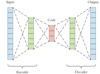autoencoder, AE

1987年 

Yann LeCun 

• 自编码器
千次阅读
2019-07-01 15:36:08

# 自编码器

在网上一直在搜自编码器的相关资料，但好多看不懂，可能是自己水平限制吧，毕竟对自编码器什么都不懂。经过自己这几天搜集资料，希望能够写一篇相对完善的关于自编码器的资料，希望能有小白看过之后对自编码器有一个初步的了解。

自编码器是神经网络的一种，经过训练后能够将输入复制到输出。该网络可以看到由两部分组成：一个由函数h=f(x)表示的编码器和一个生成重构的解码器r=g(h)。这个可能很多人认为没有什么作用，但是我们需要的就是对自编码器进行训练得到中间层数据，多应用于降维。这个需要限制h的维度比x小，这种编码维度小于输入维度的自编码器成为欠完备自编码器。

## 1.欠完备自编码器

欠完备自编码器的学习过程可以简单的描述为最小化一个损失函数L(x,g(f(x)))，其中L是一个损失函数。当编码器是线性且L是均方误差，欠完备的自编码器就会学习出与PCA相同的生成子空间。这种情况下，自编码器在训练来执行复制任务的同时学到了训练数据的主元子空间。因此拥有非线性编码器函数f和非线性解码器函数g的自编码器能够学习出更强大的PCA非线性推广。

## 2.正则自编码器

理想情况下，根据要建模的数据分布的复杂性，选择合适的编码维数和编码器、解码器容量，就可以成功训练任意架构的自编码器。正则自编码器就可以担当重任。正则编码器使用的损失函数可以鼓励模型学习其他特性（除了将模型复制到输出），而不必限制使用浅层的编码器和解码器以及小的编码维数来限制模型的容量。这些特性包括稀疏表示、表示的小导数以及对噪声或输入缺失的鲁棒性。即使模型容量大到足以学习一个无意义的恒等函数，非线性且过完备的正则自编码器仍然能够从数据中学到一些关于数据分布的有用信息。

强调与自编码器联系的两个生成式建模方法是Helmholtz机的衍生模型，如变分自编码器和生成随机网络。这些变种自编码器能够学习出高容量且过完备的模型，进而发现输入数据中有用的结构信息，并且也无须对模型进行正则化。

### 2.1 稀疏自编码器

稀疏自编码器简单的在训练时结合编码层的系数惩罚Ω(h)和重构误差：

L ( x , g ( f ( x ) ) ) + Ω ( h ) {\rm{L}}(x,g(f(x))) + \Omega (h)

其中g(h)是解码器的输出，通常h是编码器的输出，即h=f(x)。

稀疏自编码器一般用来学习特征，一边用于像分类这样的任务。这里可以将惩罚项Ω(h)视为加到前馈网络的正则项，这个前馈网络的主要任务是将输入复制到输出（无监督学习的目标），并尽可能地根据这些稀疏特征执行一些监督学习任务，这样的话能得到学习有用特征的模型。这里的正则项取决于数据，而不是一个先验，不过仍然可以认为是这些正则项隐式地表达了对函数的偏好。

将稀疏自编码器视为是对带有潜变量的生成模型的近似最大似然训练，则有

p &VeryThinSpace; m o d &VeryThinSpace; e l ( x , h ) = p &VeryThinSpace; m o d &VeryThinSpace; e l ( h ) p &VeryThinSpace; m o d &VeryThinSpace; e l ( x ∣ h ) {\rm{p}}_{\bmod {\rm{el}}} (x,h) = p_{\bmod el} (h)p_{\bmod el} (x|h)

p &VeryThinSpace; m o d &VeryThinSpace; e l ( h ) p_{\bmod el} (h) 视为模型关于潜变量的先验分布，表示模型看到x的信念先验。注意此处的先验和之前的先验并不一样，之前指的是看到数据前就对模型参数的先验进行编码。对数似然函数可分解为：

log ⁡ p &VeryThinSpace; m o d &VeryThinSpace; e l ( x ) = log ⁡ ∑ h p &VeryThinSpace; m o d &VeryThinSpace; e l ( h , x ) \log p_{\bmod el} (x) = \log \sum\limits_h {p_{\bmod el} (h,x)}

可以认为自编码器使用一个高似然值h的点估计近似这个总和。因此估计这个总和就转化为寻找一个高似然值h。由于

log ⁡ p &VeryThinSpace; m o d &VeryThinSpace; e l ( h , x ) = log ⁡ p &VeryThinSpace; m o d &VeryThinSpace; e l ( h ) + log ⁡ p &VeryThinSpace; m o d &VeryThinSpace; e l ( x ∣ h ) \log p_{\bmod el} (h,x) = \log p_{\bmod el} (h) + \log p_{\bmod el} (x|h)

log ⁡ p &VeryThinSpace; m o d &VeryThinSpace; e l ( h ) \log p_{\bmod el} (h) 项能被稀疏诱导。如Laplace先验，

p &VeryThinSpace; m o d &VeryThinSpace; e l ( h i ) = λ 2 e − λ ∣ h i ∣ p_{\bmod el} (h_i ) = \frac{\lambda }{2}e^{ - \lambda \left| {h_i } \right|}

对应于绝对值稀疏惩罚。将对数先验表示为绝对值惩罚，得到：

Ω ( h ) = λ ∑ i ∣ h i ∣ \Omega (h) = \lambda \sum\limits_i {\left| {h_i } \right|}

$$• \log p_{\bmod el} (h) = \sum\limits_i {(\lambda \left| {h_i } \right| - \log \frac{\lambda }{2})} = \Omega (h) + const$$

这里的常数项只和λ有关。从稀疏性导致 p &VeryThinSpace; m o d &VeryThinSpace; e l ( h ) p_{\bmod el} (h) 学习成近似最大似然的结果看，稀疏惩罚完全不是一个正则项。这仅仅影响模型关于潜变量的分布。同时这也是为什么自编码器学习到的特征是有用的另一个解释：它们描述的潜变量可以解释输入。

### 2.2 去噪自编码器

除了像之前稀疏自编码器向代价函数增加一个惩罚项，也可以通过改变重构误差项来获得一个能学到有用信息的自编码器。
去噪自编码器（denoising autoencoder,DAE）是一类接受损坏数据作为输入，并训练来预测原始未被损坏数据作为输出的自编码器。损失函数为
L ( x , g ( f ( x ~ ) ) ) L(x,g(f(\tilde x))) ,即

L = − log ⁡ p d e c o d e r ( x ∣ h = f ( x ~ ) ) L = - \log p_{decoder} (x|h = f(\tilde x))

DAE的训练过程如下图所示。引入一个损坏过程 C ( x ~ ∣ x ) C({\rm{\tilde x|x}}) ,这个条件分布代表给定数据样本x产生损坏样本 的概率。自编码器则根据以下过程，从训练数据对 ( x , x ~ ) (x,\tilde x) 中学习重构分布 p r e c o n s t r u c t ( x ∣ x ~ ) p_{reconstruct} ({\rm{x|\tilde x)}} :

1. 从训练数据中采集一个训练样本 ；

2. C ( x ~ ∣ x = x ) C({\rm{\tilde x}}|{\rm{x}} = x)
采一个损坏样本;

3. ( x , x ~ ) (x,\tilde x) 作为训练样本来估计自编码器的重构分布 p r e c o n s t r u c t ( x ∣ x ~ ) = p d e c o d e r ( x ∣ h ) p_{reconstruct} (x|\tilde x) = p_{decoder} (x|h)
，其中 h h 是编码器 f ( x ~ ) f(\tilde x) 的输出, p d e c o d e r p_{decoder}
根据解码函数 g ( h ) g(h) 定义。DAE的一个重要特性就是DAE的训练准则(条件高斯
p ( x ∣ h ) p(x|h)
)能让自编码器学到能估计数据分布得分的向量场 ( g ( f ( x ) ) − x ) (g(f(x)) - x) 。在一个确定的噪声水平下，正则化的得分匹配不是一致估计量，相反它会恢复分布的一个模糊版本，然而当噪声水平趋向于0且训练样本数趋向于无穷时，一致性就会恢复。其中得分是一个特定的梯度场： ∇ x log ⁡ p ( x ) \nabla _x \log p(x)

对于连续的 x x ,高斯损坏和重构分布的去噪准则得到的得分估计适用于一般编码器和解码器的参数化。这意味着一个使用平方误差准则

∥ g ( f ( x ~ ) ) − x ∥ 2 \left\| {g(f(\tilde x)) - x} \right\|^2

和噪声方差为 σ 2 \sigma ^2 的损坏

C ( x ~ = x ~ ∣ x ) = N ( x ~ ; μ = x , Σ = σ 2 I ) C({\rm{\tilde x}} = \tilde x|x) = N(\tilde x;\mu = x,\Sigma = \sigma ^2 I)

的通用编码器-解码器架构可以用来估计训练得分。

### 2.3 收缩自编码器

收缩自编码器在编码 的基础上添加了显示的正则项，鼓励 h = f ( x ) h = f(x)
的导数尽可能小：

Ω ( h ) = λ ∥ ∂ f ( x ) ∂ x ∥ F 2 \Omega (h) = \lambda \left\| {\frac{\partial f(x)}{\partial x}} \right\|_F^2

惩罚项 Ω ( h ) \Omega (h) 为平方Frobenius范数（元素平方之和），作用于与编码器的函数相关偏导数的Jacobian矩阵。
去噪自编码器和收缩自编码器之间存在一定联系：Alain and Bengio（2013）指出在小高斯噪声的限制下，当重构函数将 x x 映射到 r = g ( f ( x ) ) r = g(f(x)) 时，去噪重构误差与收缩惩罚项是等价的。换句话说，去噪自编码器能抵抗小且有限的输入扰动，而收缩自编码器使特征提取函数能抵抗极小的输入扰动。

## 3. 自编码器的深度和表示能力

深度自编码器（编码器至少包含一层额外隐藏层）在给定足够多的隐藏单元的情况下，能以任意精度近似任何从输入到编码的映射。深度可以指数地降低表示某些函数的计算成本。深度也能指数的减少学习一些函数所需的训练数据量。

训练深度自编码器的普遍策略是训练一堆浅层的自编码器来贪心地预训练相应的深度架构。所以即使最终目标是训练深度自编码器，我们也经常会遇到浅层自编码器。

## 4. 随机编码器和解码器

自编码器本质上是一个前馈网络，可以使用与传统前馈网络相同的损失函数和输出单元。
为了彻底地与前馈网络相区别，可以将编码函数 f ( x ) f(x) 的概念推广为编码分布。任何潜变量模型 p &VeryThinSpace; m o d &VeryThinSpace; e l ( h , x ) {\rm{p}}_{\bmod el} (h,x) 定义一个随机编码器

p e n c o d e r ( h ∣ x ) = p &VeryThinSpace; m o d &VeryThinSpace; e l ( h ∣ x ) p_{encoder} (h|x) = p_{\bmod el} (h|x)

以及一个随机解码器

p d e c o d e r ( x ∣ h ) = p &VeryThinSpace; m o d &VeryThinSpace; e l ( x ∣ h ) p_{decoder} (x|h) = p_{\bmod el} (x|h)

通常情况下，编码器和解码器的分布没必要是与唯一一个联合分布 p &VeryThinSpace; m o d &VeryThinSpace; e l ( x , h ) p_{\bmod el} (x,h) 相容的条件分布。Alain et al（2015）指出，在保证足够容量和样本的情况下，将编码器和解码器作为去噪自编码器训练，能使它们渐进地相容。

## 5. 预测稀疏分解

预测稀疏分解是稀疏编码和参数化自编码器的混合模型。参数化编码器被训练为能预测迭代推断的输出。这个模型由一个编码器f(x)和一个解码器g(h)组成，并且都是参数化的。在训练过程中，h由优化算法控制。优化过程是最小化

∥ x − g ( h ) ∥ 2 + λ ∣ h ∣ 1 + γ ∥ h − f ( x ) ∥ 2 \left\| {x - g(h)} \right\|^2 + \lambda \left| h \right|_1 + \gamma \left\| {h - f(x)} \right\|^2

就像稀疏编码，训练算法交替地相对h和模型的参数最小化上述目标。相对h最小化较快，因为f(x)提供h的良好初始值以及损失函数将h约束在f(x)附近。简单的梯度下降算法只需10步左右就能获得理想的h。

## 6. 总结

自编码器跟其他很多机器学习算法一样，利用了数据集中在一个低维流形或者一小组这样的流行的思想。流行的一个重要特征就是切平面的集合。d维流形上的一点x，切平面由能张成流行上允许变动的局部方向的d维基向量给出。

所有自编码器的训练过程涉及两种推动力的折衷：

1)学习训练样本x的表示h使得x能通过解码器近似地从h中恢复。

2)满足约束或正则惩罚。这既可以是限制自编码器容量的架构约束，也可以是加入到重构代价的一个正则项。

更多相关内容
• Convolutional Auto-Encoders卷积自编码器的Matlab代码，可以运行caeexamples.m对手写数据mnist_uint8进行训练测试
• 将节点相似度矩阵，作为深度稀疏自动编码器的输入，并通过不断迭代，作为输出低维特征矩阵。（matlab编写）
• 本资源是3层的自编码器加上稀疏正则项约束的matlab代码。隐层激活函数选sigmoid函数，输出层选线性函数，程序中以一个标准数据集sonar为例，使用该方法可以做无监督表征学习，数据压缩，多任务学习等表征学习
• 最先提出深度学习算法hinton的自动编码器matlab代码，内容是：利用多层rbm进行自动编码的多层特征训练，然后使用梯度算法进行fine turn。可以进行特征提取，也可以进行分类。
• 实用代码 06 变分自编码器实用代码 06 变分自编码器实用代码 06 变分自编码器实用代码 06 变分自编码器实用代码 06 变分自编码器实用代码 06 变分自编码器实用代码 06 变分自编码器实用代码 06 变分自编码器实用代码...
• 实现堆叠降噪自编码器功能，以tensorflow中的mnist数据集为例，python2.7深度学习
• 自编码器是开发无监督学习模型的主要方式之一。但什么是自动编码器？ 简而言之，自动编码器通过接收数据、压缩和编码数据，然后从编码表示中重构数据来进行操作。对模型进行训练，直到损失最小化并且尽可能接近地...

# 一、什么是自动编码器？

自编码器是开发无监督学习模型的主要方式之一。但什么是自动编码器？

简而言之，自动编码器通过接收数据、压缩和编码数据，然后从编码表示中重构数据来进行操作。对模型进行训练，直到损失最小化并且尽可能接近地再现数据。通过这个过程，自动编码器可以学习数据的重要特征。

自动编码器是由多个层组成的神经网络。自动编码器的定义方面是输入层包含与输出层一样多的信息。输入层和输出层具有完全相同数量的单元的原因是自动编码器旨在复制输入数据。然后分析数据并以无监督方式重建数据后输出数据副本。

通过自动编码器的数据不仅仅是从输入直接映射到输出。自动编码器包含三个组件：压缩数据的编码（输入）部分、处理压缩数据（或瓶颈）的组件和解码器（输出）部分。当数据被输入自动编码器时，它会被编码，然后压缩到更小的尺寸。然后对网络进行编码/压缩数据的训练，并输出该数据的重建。

神经网络学习了输入数据的“本质”或最重要的特征，这是自动编码器的核心价值。训练完网络后，训练好的模型就可以合成相似的数据，并添加或减去某些目标特征。例如，您可以在加了噪声的图像上训练自动编码器，然后使用经过训练的模型从图像中去除噪声。

自编码器的应用包括：异常检测、数据去噪（例如图像、音频）、图像着色、图像修复、信息检索等、降维等

# 二、自动编码器的架构

自动编码器基本上可以分为三个不同的组件：编码器、瓶颈和解码器。

编码器：编码器是一个前馈、全连接的神经网络，它将输入压缩为潜在空间表示，并将输入图像编码为降维的压缩表示。压缩后的图像是原始图像的变形版本。

code：网络的这一部分包含输入解码器的简化表示。

解码器：解码器和编码器一样也是一个前馈网络，结构与编码器相似。该网络负责将输入从代码中重建回原始维度。

首先，输入通过编码器进行压缩并存储在称为code的层中，然后解码器从代码中解压缩原始输入。自编码器的主要目标是获得与输入相同的输出。

通常情况下解码器架构是编码器的镜像，但也不是绝对的。唯一的要求是输入和输出的维度必须相同。

# 三、自动编码器的类型

## 1、卷积自动编码器

卷积自动编码器是通用的特征提取器。卷积自编码器是采用卷积层代替全连接层，原理和自编码器一样，对输入的象征进行降采样以提供较小维度潜在表示，并强制自编码器学习象征的压缩版本。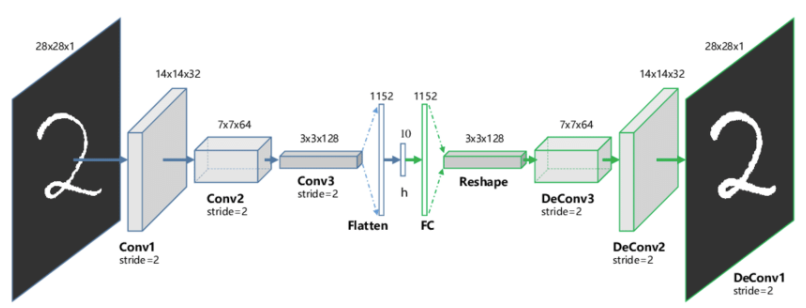## 2、去噪自动编码器

这种类型的自动编码器适用于部分损坏的输入，并训练以恢复原始未失真的图像。如上所述，这种方法是限制网络简单复制输入的有效方法。

目标是网络将能够复制图像的原始版本。通过将损坏的数据与原始数据进行比较，网络可以了解数据的哪些特征最重要，哪些特征不重要/损坏。换句话说，为了让模型对损坏的图像进行去噪，它必须提取图像数据的重要特征。## 3、收缩自动编码器

收缩自动编码器的目标是降低表示对训练输入数据的敏感性。 为了实现这一点，在自动编码器试图最小化的损失函数中添加一个正则化项或惩罚项。

收缩自动编码器通常仅作为其他几种自动编码器节点存在。去噪自动编码器使重建函数抵抗输入的小但有限大小的扰动，而收缩自动编码器使特征提取函数抵抗输入的无穷小扰动。

## 4、变分自动编码器

Variational Autoencoders，这种类型的自动编码器对潜在变量的分布做出了假设，并在训练过程中使用了随机梯度变分贝叶斯估计器。

训练时，编码器为输入图像的不同特征创建潜在分布。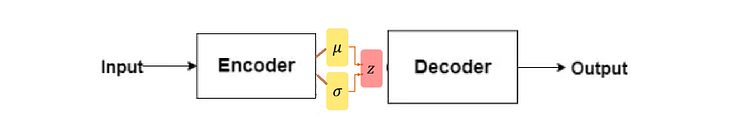本质上，该模型学习了训练图像的共同特征，并为它们分配了它们发生的概率。然后可以使用概率分布对图像进行逆向工程，生成与原始训练图像相似的新图像。

这种类型的自动编码器可以像GAN一样生成新图像。由于 VAE 在生成行为方面比GAN更加灵活和可定制，因此它们适用于任何类型的艺术生成。

# 四、自动编码器与PCA有何不同？

PCA 和自动编码器是降低特征空间维数的两种流行方法。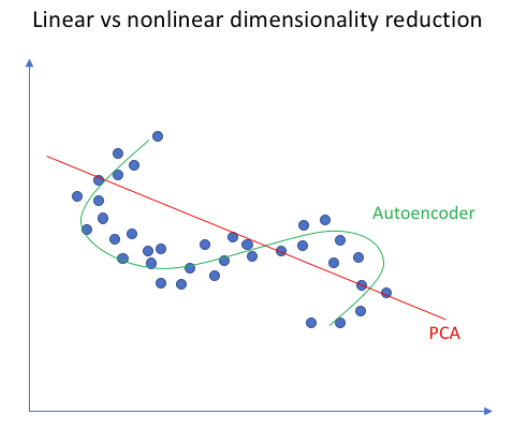PCA 从根本上说是一种线性变换，但自动编码器可以描述复杂的非线性过程。如果我们要构建一个线性网络（即在每一层不使用非线性激活函数），我们将观察到与 PCA 中相似的降维。

PCA 试图发现描述原始数据的低维超平面，而自动编码器能够学习非线性流形（流形简单地定义为连续的、不相交的表面）。

与自动编码器相比，PCA 的计算速度更快且成本更低。但由于参数数量多，自编码器容易过拟合。

# 五、自编码器与GAN有何不同？

1、两者都是生成模型。AE试图找到以特定输入（更高维度）为条件的数据的低维表示，而GAN 试图创建足以概括以鉴别器为条件的真实数据分布的表示。

2、虽然它们都属于无监督学习的范畴，但它们是解决问题的不同方法。

GAN 是一种生成模型——它应该学习生成数据集的新样本

变分自动编码器是生成模型，但普通的自动编码器只是重建它们的输入，不能生成真实的新样本。

https://www.quora.com/What-is-the-difference-between-Generative-Adversarial-Networks-and-Autoencoders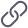https://www.quora.com/What-is-the-difference-between-Generative-Adversarial-Networks-and-Autoencoders

# 六、实例1：去噪自动编码器

## 1、概述

去噪自动编码器的一个应用的例子是预处理图像以提高光学字符识别 (OCR) 算法的准确性。如果您以前应用过OCR，就会知道一丁点错误的噪声（例如，打印机墨水污迹、扫描过程中的图像质量差等）都会严重影响OCR识别的效果。使用去噪自编码器，可以自动对图像进行预处理，提高质量，从而提高OCR识别算法的准确性。

我们这里故意向MNIST训练图像添加噪声。目的是使我们的自动编码器能够有效地从输入图像中去除噪声。

## 2、参考代码

创建autoencoder_for_denoising.py文件，插入以下代码。

# import the necessary packages
from tensorflow.keras.layers import BatchNormalization
from tensorflow.keras.layers import Conv2D
from tensorflow.keras.layers import Conv2DTranspose
from tensorflow.keras.layers import LeakyReLU
from tensorflow.keras.layers import Activation
from tensorflow.keras.layers import Flatten
from tensorflow.keras.layers import Dense
from tensorflow.keras.layers import Reshape
from tensorflow.keras.layers import Input
from tensorflow.keras.models import Model
from tensorflow.keras import backend as K
import numpy as np

class ConvAutoencoder:
@staticmethod
def build(width, height, depth, filters=(32, 64), latentDim=16):
# initialize the input shape to be "channels last" along with
# the channels dimension itself
# channels dimension itself
inputShape = (height, width, depth)
chanDim = -1
# define the input to the encoder
inputs = Input(shape=inputShape)
x = inputs

# loop over the number of filters
for f in filters:
# apply a CONV => RELU => BN operation
x = Conv2D(f, (3, 3), strides=2, padding="same")(x)
x = LeakyReLU(alpha=0.2)(x)
x = BatchNormalization(axis=chanDim)(x)
# flatten the network and then construct our latent vector
volumeSize = K.int_shape(x)
x = Flatten()(x)
latent = Dense(latentDim)(x)
# build the encoder model
encoder = Model(inputs, latent, name="encoder")

# start building the decoder model which will accept the
# output of the encoder as its inputs
latentInputs = Input(shape=(latentDim,))
x = Dense(np.prod(volumeSize[1:]))(latentInputs)
x = Reshape((volumeSize, volumeSize, volumeSize))(x)
# loop over our number of filters again, but this time in
# reverse order
for f in filters[::-1]:
# apply a CONV_TRANSPOSE => RELU => BN operation
x = Conv2DTranspose(f, (3, 3), strides=2, padding="same")(x)
x = LeakyReLU(alpha=0.2)(x)
x = BatchNormalization(axis=chanDim)(x)
# apply a single CONV_TRANSPOSE layer used to recover the
# original depth of the image
x = Conv2DTranspose(depth, (3, 3), padding="same")(x)
outputs = Activation("sigmoid")(x)
# build the decoder model
decoder = Model(latentInputs, outputs, name="decoder")
# our autoencoder is the encoder + decoder
autoencoder = Model(inputs, decoder(encoder(inputs)), name="autoencoder")
# return a 3-tuple of the encoder, decoder, and autoencoder
return (encoder, decoder, autoencoder)

# set the matplotlib backend so figures can be saved in the background
import matplotlib
matplotlib.use("Agg")
# import the necessary packages
from tensorflow.keras.datasets import mnist
import matplotlib.pyplot as plt
import numpy as np
import argparse
import cv2
# construct the argument parse and parse the arguments
ap = argparse.ArgumentParser()
ap.add_argument("-s", "--samples", type=int, default=8, help="# number of samples to visualize when decoding")
ap.add_argument("-o", "--output", type=str, default="output.png", help="path to output visualization file")
ap.add_argument("-p", "--plot", type=str, default="plot.png", help="path to output plot file")
args = vars(ap.parse_args())

# initialize the number of epochs to train for and batch size
EPOCHS = 25
BS = 32
((trainX, _), (testX, _)) = mnist.load_data()
# add a channel dimension to every image in the dataset, then scale
# the pixel intensities to the range [0, 1]
trainX = np.expand_dims(trainX, axis=-1)
testX = np.expand_dims(testX, axis=-1)
trainX = trainX.astype("float32") / 255.0
testX = testX.astype("float32") / 255.0

# sample noise from a random normal distribution centered at 0.5 (since
# our images lie in the range [0, 1]) and a standard deviation of 0.5
trainNoise = np.random.normal(loc=0.5, scale=0.5, size=trainX.shape)
testNoise = np.random.normal(loc=0.5, scale=0.5, size=testX.shape)
trainXNoisy = np.clip(trainX + trainNoise, 0, 1)
testXNoisy = np.clip(testX + testNoise, 0, 1)

# construct our convolutional autoencoder
print("[INFO] building autoencoder...")
(encoder, decoder, autoencoder) = ConvAutoencoder.build(28, 28, 1)
autoencoder.compile(loss="mse", optimizer=opt)
# train the convolutional autoencoder
H = autoencoder.fit(trainXNoisy, trainX, validation_data=(testXNoisy, testX), epochs=EPOCHS, batch_size=BS)
# construct a plot that plots and saves the training history
N = np.arange(0, EPOCHS)
plt.style.use("ggplot")
plt.figure()
plt.plot(N, H.history["loss"], label="train_loss")
plt.plot(N, H.history["val_loss"], label="val_loss")
plt.title("Training Loss and Accuracy")
plt.xlabel("Epoch #")
plt.ylabel("Loss/Accuracy")
plt.legend(loc="lower left")
plt.savefig(args["plot"])

# use the convolutional autoencoder to make predictions on the
# testing images, then initialize our list of output images
print("[INFO] making predictions...")
decoded = autoencoder.predict(testXNoisy)
outputs = None
# loop over our number of output samples
for i in range(0, args["samples"]):
# grab the original image and reconstructed image
original = (testXNoisy[i] * 255).astype("uint8")
recon = (decoded[i] * 255).astype("uint8")
# stack the original and reconstructed image side-by-side
output = np.hstack([original, recon])
# if the outputs array is empty, initialize it as the current
# side-by-side image display
if outputs is None:
outputs = output
# otherwise, vertically stack the outputs
else:
outputs = np.vstack([outputs, output])
# save the outputs image to disk
cv2.imwrite(args["output"], outputs)

然后输入以下命令进行训练。

python train_denoising_autoencoder.py --output output_denoising.png --plot plot_denoising.png

## 3、训练结果

经过25epoch的训练结果。

对应的去噪结果图，左边是添加噪声的原始MNIST数字，而右边是去噪自动编码器的输出——可以看到去噪自动编码器能够在消除噪音的同时从图像中恢复原始信号。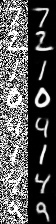展开全文神经网络 机器学习 无监督学习
• 一、 自编码器（Auto-Encoder）介绍 1. AE算法的原理 2. AE算法的作用 3. AE算法的优缺点 二、 自编码器AutoEncoder解决异常检测问题（手把手写代码） 1. 关于数据集准备 2. 数据预处理 3. 建立自编码模型 4. 训练...

### 一、 自编码器（Auto-Encoder）介绍

#### 1. AE算法的原理

Auto-Encoder，中文称作自编码器，是一种无监督式学习模型。它基于反向传播算法与最优化方法（如梯度下降法），利用输入数据 X X 本身作为监督，来指导神经网络尝试学习一个映射关系，从而得到一个重构输出 X R X^R 。在时间序列异常检测场景下，异常对于正常来说是少数，所以我们认为，如果使用自编码器重构出来的输出 X R X^R 跟原始输入的差异超出一定阈值（threshold）的话，原始时间序列即存在了异常。

通过算法模型包含两个主要的部分：Encoder（编码器）和Decoder（解码器）

编码器的作用是把高维输入 X X 编码成低维的隐变量 h h 从而强迫神经网络学习最有信息量的特征；解码器的作用是把隐藏层的隐变量 h h 还原到初始维度，最好的状态就是解码器的输出能够完美地或者近似恢复出原来的输入, 即 X R X^R ≈ X ≈X .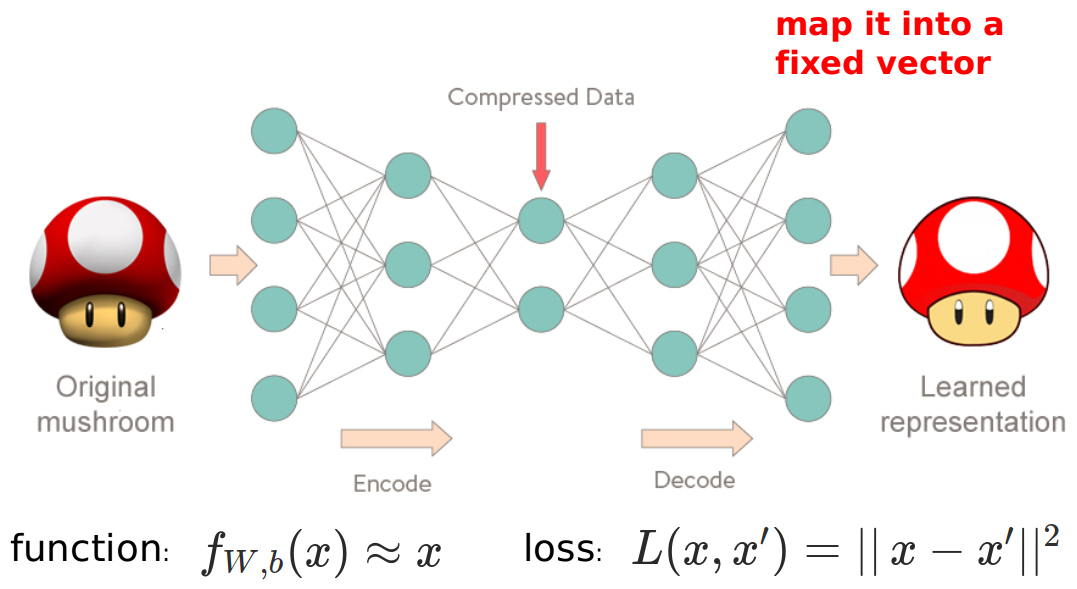如图所示，

(1)从输入层 ->隐藏层的原始数据X的编码过程：
h = g θ 1 ( x ) = σ ( W 1 x + b 1 ) h=g\theta _1(x)=\sigma (W_1x+b_1)

(2)从隐藏层 -> 输出层的解码过程：
x ^ = g θ 2 ( h ) = σ ( W 2 x + b 2 ) \hat{x} =g\theta _2(h)=\sigma (W_2x+b_2)

那么算法的优化目标函数就写为： M i n i m i z e L o s s = d i s t ( X , X R ) MinimizeLoss=dist(X,X^R)
其中 d i s t dist 为二者的距离度量函数，通常用 MSE（均方方差）

#### 2. AE算法的作用

自编码可以实现类似于 PCA 等数据降维、数据压缩的特性。从上面自编码的网络结构图，如果输入层神经元的个数 n 大于隐层神经元个数 m，那么我们就相当于把数据从 n 维降到了 m 维；然后我们利用这 m 维的特征向量，进行重构原始的数据。这个跟 PCA 降维一模一样，只不过 PCA 是通过求解特征向量，进行降维，是一种线性的降维方式，而自编码是一种非线性降维。自编码器常用于异常检测和状态检测

#### 3. AE算法的优缺点

优点：泛化性强，无监督不需要数据标注

缺点：针对异常识别场景，训练数据需要为正常数据。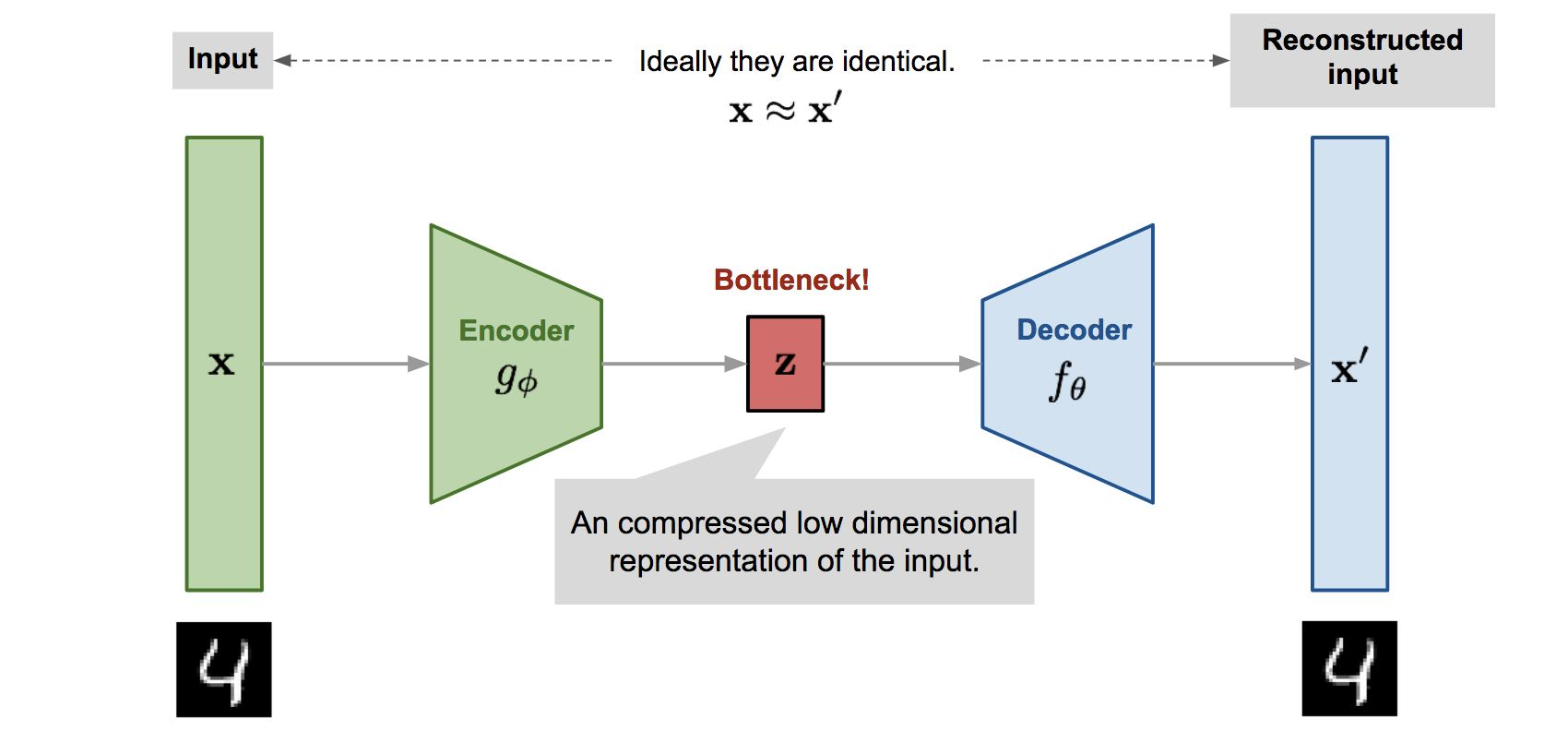### 二、 自编码器AutoEncoder解决异常检测问题（手把手写代码）

#### 1. 关于数据集准备

从NASA声学和振动数据库下载数据集。
点击直达👉Kaggle官网
点击直达👉百度网盘链接

原始数据集中包含三组测试，每组测试都是包含很多文件，这些文件都是10分钟的数据量（测试1略有例外），采样周期为1s，采样率设置为20 kHz，所以每个文件由20480点组成。文件名为采集数据的起始时间戳，数据文件中的每个记录（行）是一次采样点，每一列表示一个测试通道（NI采集的卡的测试通道）。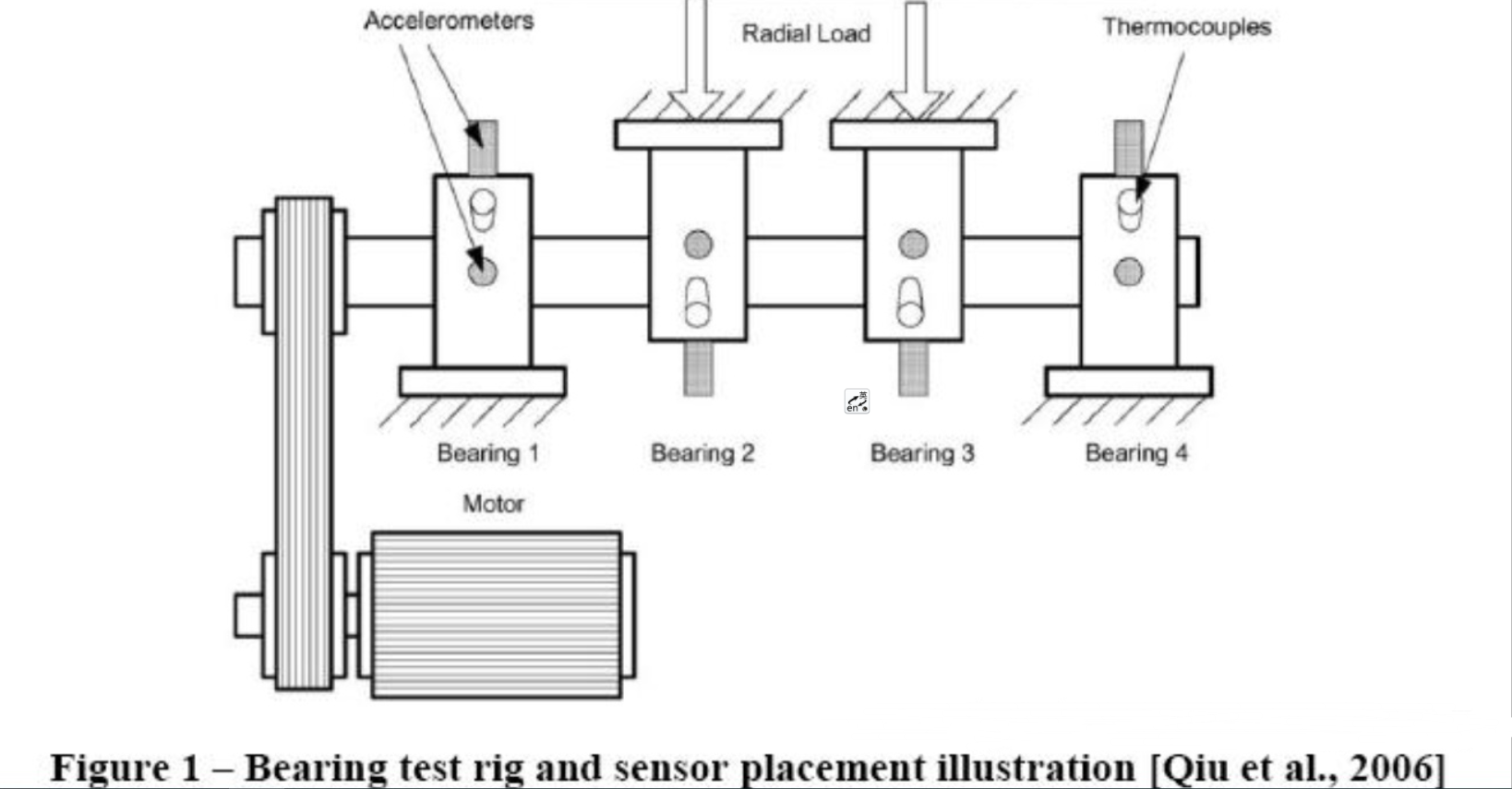载入数据集

# Import libraries, core libraries are numpy and sklearn
import os
import pandas as pd
import numpy as np
import tensorflow as tf
from sklearn import preprocessing
import seaborn as sns
sns.set(color_codes=True)
import matplotlib.pyplot as plt
# sns.axes_style("white")
# sinplot()
# sns.despine()

data_dir = folder+'\\2nd_test'
merged_data = pd.DataFrame()

for filename in os.listdir(data_dir):
#     print(filename)
dataset_mean_abs = np.array(dataset.abs().mean())
dataset_mean_abs = pd.DataFrame(dataset_mean_abs.reshape(1,4))
dataset_mean_abs.index = [filename]
merged_data = merged_data.append(dataset_mean_abs)
merged_data.reset_index(inplace=True)  # reset index to get datetime as columns

# 将原始数据集聚合为单个csv文件
merged_data.columns = ['Datetime','Bearing 1','Bearing 2','Bearing 3','Bearing 4'] # rename columns
merged_data.sort_values(by='Datetime',inplace=True)
merged_data.to_csv('2nd_test_resmaple_10minutes.csv')

# 读取数据集
merged_data = pd.read_csv('2nd_test_resmaple_10minutes.csv',index_col='Datetime',usecols=['Datetime','Bearing 1','Bearing 2','Bearing 3','Bearing 4'])
merged_data.index = pd.to_datetime(merged_data.index, format='%Y.%m.%d.%H.%M.%S')
merged_data.plot()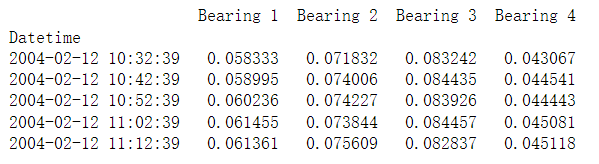#### 2. 数据预处理

对于自编码器（深度学习神经网络）来说，至少要进行两个预处理：

• 划分训练集和测试集
• 归一化操作

划分训练集和测试集，可以帮助我们验证自己的模型，并且让模型更加鲁棒。对于异常诊断来说，需要将正常数据放在训练集中。​对于时间序列，我们直接按照时间点进行切分，这里选取2004-02-13 23:52:39。

归一化操作对于神经网络来说至关重要，因为没有归一化，会导致算法无法快速进行梯度下降，在“有生之年”（有限的迭代次数内）无法达到最优解。

# Data pre-processing
# Split the training and test sets using 2004-02-13 23:52:39 as the cut point
dataset_train = merged_data['2004-02-12 11:02:39':'2004-02-13 23:52:39']
dataset_test  = merged_data['2004-02-13 23:52:39':]
dataset_train.plot(figsize = (12,6))

"""
Normalize data
"""
scaler = preprocessing.MinMaxScaler() # 归一化

X_train = pd.DataFrame(scaler.fit_transform(dataset_train), # Find the mean and standard deviation of X_train and apply them to X_train
columns=dataset_train.columns,
index=dataset_train.index)

# Random shuffle training data
X_train.sample(frac=1)

X_test = pd.DataFrame(scaler.transform(dataset_test),
columns=dataset_test.columns,
index=dataset_test.index)


#### 3. 建立自编码模型

创建深度神经网络，较为常用的是Keras （高阶API）以及Tensorflow( 作为Backend），本文即是采用 Tensorflow

自编码模型一般的神经网络，其内部结构呈现一定对称性。这里我们创建三层神经网络：第一层有10个节点（编码器），中间层有2个节点（编码），第三层有10个节点（解码器）。

一个较好的习惯是，对于神经网络总是从简单模型开始尝试。所以这个三层神经网络会是一个比较好的开始。

激活函数，一般会优先选择“Relu”，当“Relu”无法满足需求的时候，再去考虑其他激活函数，比如Relu的一些衍生版本。这里推荐的激活函数是“Elu”，它是Relu的其中一个优化版本，可以避免出现神经元无法更新的情况。

# Build AutoEncoding model
def AutoEncoder_build(model, X_train, act_func):
tf.random.set_seed(10)

# act_func = 'elu'

# Input layer:
model = tf.keras.Sequential() # Sequential() is a container that describes the network structure of the neural network, sequentially processing the model

# First hidden layer, connected to input vector X.
kernel_initializer='glorot_uniform', # Weight initialization
kernel_regularizer=tf.keras.regularizers.l2(0.0), # Regularization to prevent overfitting
input_shape=(X_train.shape,)
)
)

kernel_initializer='glorot_uniform'))

kernel_initializer='glorot_uniform'))

kernel_initializer='glorot_uniform'))

print(model.summary())
tf.keras.utils.plot_model(model, show_shapes=True)

return model

model = tf.keras.Sequential()
model = AutoEncoder_build(model=model,X_train=X_train, act_func='elu')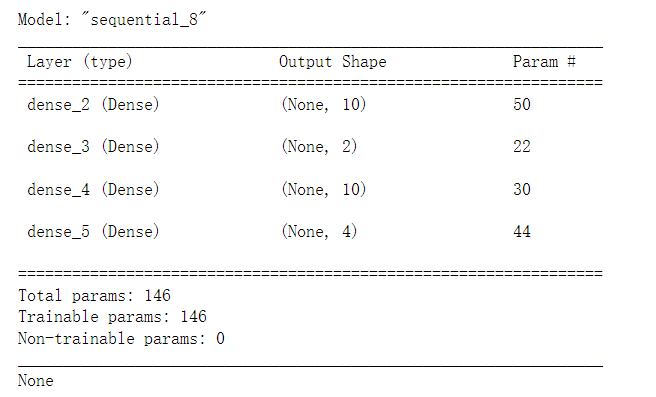#### 4. 训练模型

训练模型很简单，只需要调用 fit 函数。需要注意的是，对于自编码器来说，输入和输出都是X_train 特征。 另外我们划分出 5% 的数据集作为验证集来验证模型的泛化性。

def AutoEncoder_main(model,Epochs,BATCH_SIZE,validation_split):
# Train model for 100 epochs, batch size of 10:
# noise
factor = 0.5
X_train_noise = X_train + factor * np.random.normal(0,1,X_train.shape)
X_train_noise = np.clip(X_train_noise,0.,1.)

history=model.fit(np.array(X_train_noise),np.array(X_train),
batch_size=BATCH_SIZE,
epochs=Epochs,
shuffle=True,
validation_split=validation_split, # Training set ratio
#                       validation_data=(X_train,X_train), # Validation set
verbose = 1)

return history

# Figure
def plot_AE_history(history):
plt.plot(history.history['loss'],
'b',
label='Training loss')
plt.plot(history.history['val_loss'],
'r',
label='Validation loss')
plt.legend(loc='upper right')
plt.xlabel('Epochs')
plt.ylabel('Loss, [mse]')
plt.ylim([0,.1])
plt.show()

history = AutoEncoder_main(model=model,Epochs=100,BATCH_SIZE=10,validation_split=0.05)

plot_AE_history(history)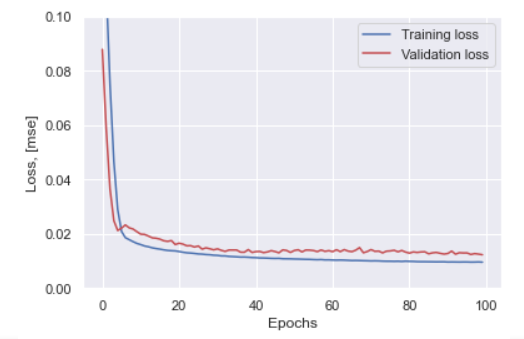当我们查看训练的loss历史曲线时，发现训练集和验证集都很好的进行拟合，而且训练集并没有出现“反弹”，也就是没有过拟合的现象。

#### 5. 查看模型的“认知水平”

当自编码器训练好后，它应该能够学习到原始数据集的内在编码（用很少的维度，比如本案例中为2），然后根据学习到的编码，在一定程度内还原原始数据集。我们可以查看还原的误差分布如何。

X_pred = model.predict(np.array(X_train))
X_pred = pd.DataFrame(X_pred,
columns=X_train.columns)
X_pred.index = X_train.index

scored = pd.DataFrame(index=X_train.index)
scored['Loss_mae'] = np.mean(np.abs(X_pred-X_train), axis = 1)
plt.figure()
sns.distplot(scored['Loss_mae'],
bins = 10,
kde= True,
color = 'blue')
plt.xlim([0.0,.5])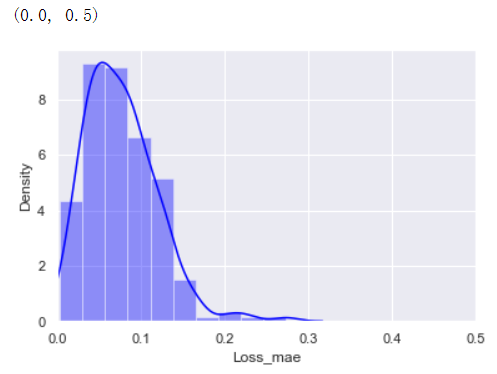因为该自编码器学习到了“正常数据”的编码格式，所以当一个数据集提供给该自编码器时，它会按照“正常数据”的编码格式去编码和解码。**如果解码后的数据集和输入数据集的误差在一定范围内，则表明输入的数据集是“正常的”，否则是“异常的"。**所以根据上图，我们知道”正常的数据“编码和解码后的误差分布在0.3以内，所以我们可以认为如果一个新的数据集编码，解码之后的误差超出该范围则为异常数据。

#### 6. 对测试集进行异常诊断

基于上面的分析，我们可以确定阈值。接下来我们仅仅需要对测试数据集进行预测，然后将预测的结果误差和阈值进行比对，确认是否为正常数据。

以下代码分别对训练集和测试集进行预测，并且计算解码误差。将误差和阈值对比后，画出趋势图。

X_pred = model.predict(np.array(X_test))
X_pred = pd.DataFrame(X_pred,
columns=X_test.columns)
X_pred.index = X_test.index

threshod = 0.3
scored = pd.DataFrame(index=X_test.index)
scored['Loss_mae'] = np.mean(np.abs(X_pred-X_test), axis = 1)
scored['Threshold'] = threshod
scored['Anomaly'] = scored['Loss_mae'] > scored['Threshold']

X_pred_train = model.predict(np.array(X_train))
X_pred_train = pd.DataFrame(X_pred_train,
columns=X_train.columns)
X_pred_train.index = X_train.index

scored_train = pd.DataFrame(index=X_train.index)
scored_train['Loss_mae'] = np.mean(np.abs(X_pred_train-X_train), axis = 1)
scored_train['Threshold'] = threshod
scored_train['Anomaly'] = scored_train['Loss_mae'] > scored_train['Threshold']
scored = pd.concat([scored_train, scored])

scored.plot(logy=True,  figsize = (10,6), ylim = [1e-2,1e2], color = ['blue','red'])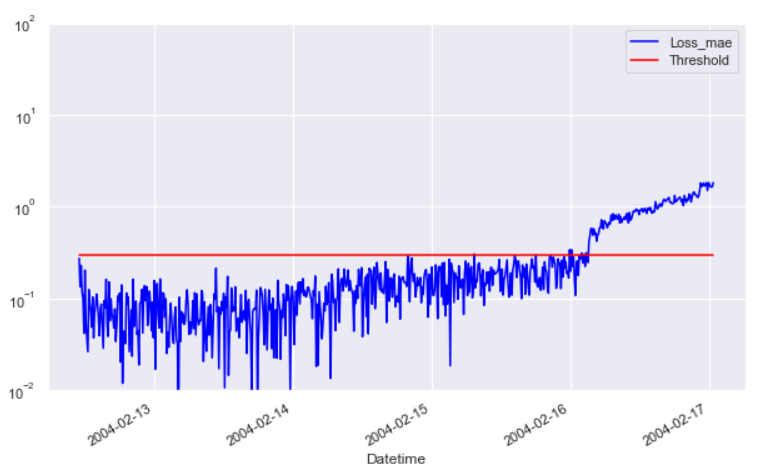可以看到，该方法还是很明显的区分出了正常数据集和异常数据集。对于正常部分来说，可能会有一些”虚假警告“，但是由于其持续时间不长，因此不会给现实的应用造成很大的困扰。因为相比于”提前三天“预警轴承的失效，短短十分钟或者二十分钟的虚假警告，可以通过double check来避免过早行动。

#### 7. 测试

① 获得encoder (功能可以把一张图像压缩为一个向量)

#获得encoder (功能可以把一张图像压缩为一个向量)
encode = tf.keras.Model(inputs=input_dim, outputs=en)
#获得decoder（把一个向量还原回图像)
input_decoder = tf.keras.layers.Input(shape=(hidden_size,))
output__decoder = model.layers[-1](input_decoder)

decode = tf.keras.Model(inputs=input_decoder, outputs=output_decoder)


② 调用encoder把测试集数据压缩为32维向量

#调用encoder把测试集数据压缩为32维向量
#encode_test = encode (x_test) # encode. predict(x_test)可以返回numpy数据(10000, 32)
encode_test = encode. predict(x_test)
encode_test.shape


③ 调用decoder把向量解码回图像

#调用decoder把向量解码回图像
# decode_test = decode (encode_test)
decode_test = decode. predict(encode_test)decode_test.shape
x_test = x_test. numpy ()


#### 8. 完整代码

# Import libraries, core libraries are numpy and sklearn
import os
import pandas as pd
import numpy as np
import tensorflow as tf
from sklearn import preprocessing
import seaborn as sns
sns.set(color_codes=True)
import matplotlib.pyplot as plt

data_dir = folder+'\\2nd_test'
merged_data = pd.DataFrame()

for filename in os.listdir(data_dir):
#     print(filename)
dataset_mean_abs = np.array(dataset.abs().mean())
dataset_mean_abs = pd.DataFrame(dataset_mean_abs.reshape(1,4))
dataset_mean_abs.index = [filename]
merged_data = merged_data.append(dataset_mean_abs)
merged_data.reset_index(inplace=True)  # reset index to get datetime as columns

# 将原始数据集聚合为单个csv文件
merged_data.columns = ['Datetime','Bearing 1','Bearing 2','Bearing 3','Bearing 4'] # rename columns
merged_data.sort_values(by='Datetime',inplace=True)
merged_data.to_csv('2nd_test_resmaple_10minutes.csv')

# 读取数据集
merged_data = pd.read_csv('2nd_test_resmaple_10minutes.csv',index_col='Datetime',usecols=['Datetime','Bearing 1','Bearing 2','Bearing 3','Bearing 4'])
merged_data.index = pd.to_datetime(merged_data.index, format='%Y.%m.%d.%H.%M.%S')
merged_data.plot()

# Data pre-processing
# Split the training and test sets using 2004-02-13 23:52:39 as the cut point
dataset_train = merged_data['2004-02-12 11:02:39':'2004-02-13 23:52:39']
dataset_test  = merged_data['2004-02-13 23:52:39':]
dataset_train.plot(figsize = (12,6))

"""
Normalize data
"""
scaler = preprocessing.MinMaxScaler() # 归一化

X_train = pd.DataFrame(scaler.fit_transform(dataset_train), # Find the mean and standard deviation of X_train and apply them to X_train
columns=dataset_train.columns,
index=dataset_train.index)

# Random shuffle training data
X_train.sample(frac=1)

X_test = pd.DataFrame(scaler.transform(dataset_test),
columns=dataset_test.columns,
index=dataset_test.index)
return X_train,X_test

# Build AutoEncoding model
def AutoEncoder_build(model, X_train, act_func):
tf.random.set_seed(10)

# act_func = 'elu'

# Input layer:
model = tf.keras.Sequential()  # Sequential() is a container that describes the network structure of the neural network, sequentially processing the model

# First hidden layer, connected to input vector X.
kernel_initializer='glorot_uniform',  # Weight initialization
kernel_regularizer=tf.keras.regularizers.l2(0.0),
# Regularization to prevent overfitting
input_shape=(X_train.shape,)
)
)

kernel_initializer='glorot_uniform'))

kernel_initializer='glorot_uniform'))

kernel_initializer='glorot_uniform'))

print(model.summary())
tf.keras.utils.plot_model(model, show_shapes=True)

return model

def AutoEncoder_main(model, Epochs, BATCH_SIZE, validation_split):
# Train model for 100 epochs, batch size of 10:
#     Epochs=100
#     BATCH_SIZE=10

factor = 0.5
X_train_noise = X_train + factor * np.random.normal(0,1,size=X_train.shape) # 设置噪声
history = model.fit(np.array(X_train), np.array(X_train),
batch_size=BATCH_SIZE,
epochs=Epochs,
validation_split=validation_split,  # Training set ratio
# shuffle=True,
verbose=1)
return history

def plot_AE_history(history):
plt.plot(history.history['loss'],
'b',
label='Training loss')
plt.plot(history.history['val_loss'],
'r',
label='Validation loss')
plt.legend(loc='upper right')
plt.xlabel('Epochs')
plt.ylabel('Loss, [mse]')
plt.ylim([0,.1])
plt.show()


X_train,X_test = data_load()
model = tf.keras.Sequential()
model = AutoEncoder_build(model=model, X_train=X_train, act_func='elu')
history = AutoEncoder_main(model=model,Epochs=100,BATCH_SIZE=10,validation_split=0.05)
plot_AE_history(history)


#### 9. 总结

以上我们完成了第二组数据集代码演示，我们可以用同样的方法（甚至同样的初始化参数）来对其他数据集进行异常检测。

比如第三组数据的结果：我们同样可以提前预测到轴承失效。
本文讲解了如何采用自编码器对异常进行检测，并且在NASA的轴承数据集上进行演示。本文主要讲解代码的实现思路以及一些参数选取的经验分享。最终的模型预测结果可以说是令人满意的，尤其是对于第二组和第三组的测试数据集都能达到很高的预测精度。

### 三、 附录

#### 1. 关于 os.path.join()

os.path.join()函数用于路径拼接文件路径，可以传入多个路径

① 存在以‘’/’’开始的参数，从最后一个以”/”开头的参数开始拼接，之前的参数全部丢弃。

import os

print(os.path.join('path','abc','yyy'))
# path\abc\yyy

print('1',os.path.join('aaa','/bbb','ccc.txt'))
# 1 /bbb\ccc.txt

print('1',os.path.join('/aaa','/bbb','ccc.txt'))
# 1 /bbb\ccc.txt

print('1',os.path.join('/aaa','/bbb','/ccc.txt'))
# 1 /ccc.txt

print('1',os.path.join('/aaa','bbb','ccc.txt'))
# 1 /aaa\bbb\ccc.txt


② 同时存在以‘’./’与‘’/’’开始的参数，以‘’/’为主，从最后一个以”/”开头的参数开始拼接，之前的参数全部丢弃。

>>> print('2',os.path.join('/aaa','./bbb','ccc.txt'))
2 /aaa\./bbb\ccc.txt

print('2',os.path.join('aaa','./bbb','/ccc.txt'))
# 2 /ccc.txt


③ 只存在以‘’./’开始的参数,会从”./”开头的参数的上一个参数开始拼接。

print('2',os.path.join('aaa','./bbb','ccc.txt'))
# 2 aaa\./bbb\ccc.txt


④ 注意：

path='C:/yyy/yyy_data/'
print(os.path.join(path,'/abc'))
# C:/abc

print(os.path.join(path,'abc'))
# C:/yyy/yyy_data/abc


#### 2. 关于 sns.set (Seaborn 绘图风格设置)

sns.set()设置风格
seaborn.set()函数参数：

seaborn.set(context=‘notebook’, style=‘darkgrid’, palette=‘deep’, font=‘sans-serif’, font_scale=1, color_codes=True, rc=None)


Seaborn其实是在matplotlib的基础上进行了更高级的API封装，从而使得作图更加容易，在大多数情况下使用seaborn就能做出很具有吸引力的图，为数据分析提供了很大的便利性。但是应该把Seaborn视为matplotlib的补充，而不是替代物。

从这个set()函数，可以看出，通过它我们可以设置背景色、风格、字型、字体等。我们定义一个函数，这个函数主要是生成100个0到15的变量，然后用这个变量画出6条曲线。

def sinplot(flip=2):
x = np.linspace(0, 15, 100)
for i in range(1, 6):
plt.plot(x, np.sin(x + i * .5) * (7 - i) * flip)
sns.set()
sinplot()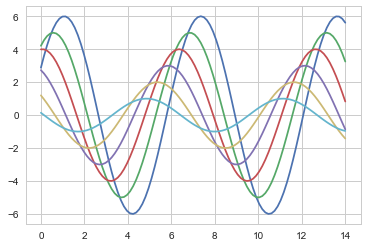#### 3. 关于 preprocessing.MinMaxScaler

preprocessing.MinMaxScaler 是归一化操作的函数，当数据(x)按照最小值中心化后，再按极差（最大值 - 最小值）缩放，数据移动了最小值个单位，并且会被收敛到 [0,1] 之间，而这个过程，就叫做数据归一化(Normalization，又称Min-Max Scaling)。注意，Normalization是归一化，不是正则化，真正的正则化是regularization，不是数据预处理的一种手段。归一化之后的数据服从正态分布，公式如下：
x ∗ = x − min ⁡ ( x ) max ⁡ ( x ) − min ⁡ ( x ) x^{*}=\frac{x-\min (x)}{\max (x)-\min (x)}
​​
在sklearn当中， 使用 preprocessing.MinMaxScaler 来实现这个功能。MinMaxScaler有一个重要参数，feature_range，控制我们希望把数据压缩到的范围，默认是[0,1] .

class sklearn.preprocessing.MinMaxScaler(feature_range=(0, 1),copy=True)


#### 4. StandardScaler类中transform和fit_transform方法有什么区别？

StandardScaler类是一个用来讲数据进行归一化和标准化的类。
所谓归一化和标准化，即应用下列公式：
X = ( x − μ ) / σ X=(x-\mu )/\sigma
答：fit_transform方法是fit和transform的结合，fit_transform(X_train) 意思是找出X_train的均值和​​​​​​​标准差，并应用在X_train上。
这时对于X_test，我们就可以直接使用transform方法。因为此时StandardScaler已经保存了X_train的均值和标准差

1. 二者的功能都是对数据进行某种统一处理（比如标准化~N(0,1)，将数据缩放(映射)到某个固定区间，归一化，正则化等）。
2. fit_transform(partData)对部分数据先拟合fit，找到该part的整体指标，如均值、方差、最大值最小值等等（根据具体转换的目的），然后对该partData进行转换transform，从而实现数据的标准化、归一化等等。
3. 根据对之前部分fit的整体指标，对剩余的数据（restData）使用同样的均值、方差、最大最小值等指标进行转换transform(restData)，从而保证part、rest处理方式相同。
4. 必须先用fit_transform(partData)，之后再transform(restData)，如果直接transform(partData)，程序会报错。
5. 如果fit_transfrom(partData)后，使用fit_transform(restData)而不用transform(restData)，虽然也能归一化，但是两个结果不是在同一个“标准”下的，具有明显差异。

#### 5. Keras kernel_initializer 权重初始化的方法

Keras kernel_initializer 权重初始化，不同的层可能使用不同的关键字来传递初始化方法，一般来说指定初始化方法的关键字是kernel_initializer 和 bias_initializer，例如：

model.add(Dense(64,
kernel_initializer='random_uniform',
bias_initializer='zeros'))


#### 6. Keras的正则化Regularizers

正则化器的概念
正则化器允许在优化过程中对层的参数或层的激活情况进行惩罚。 网络优化的损失函数也包括这些惩罚项。

tensorflow2.0内置正则项
在隐藏层中经常会使用正则来作为损失函数的惩罚项。换言之，为了约束w的可能取值空间从而防止过拟合，我们为该最优化问题加上一个约束，就是w的L1范数或者L2范数不能大于给定值。
L2正则被用于防止模型的过拟合，L1正则项被用于产生稀疏权值矩阵。
tf.keras.regularizers提供了几种内置类来提供正则。分别是class L1、class L1L2、class L2、class Regularizer 、serialize。同时提供了三个参数作为可被正则化的对象：

• kernel_regularizer : 对该层中的权值矩阵layer.weights正则
• bias_regularizer : 对该层中的偏差矩阵layer.bias正则
• activity_regularizer : 对该层的输出值矩阵layer.bias正则

调用kernel_regularizer=tf.keras.regularizers.l1_l2(l1=0.02, l2=0.01)时， l o s s e s = L 1 + L 2 = l 1 ∑ ( ∣ w i j ∣ ) + l 2 ∑ ( w i j 2 ) losses=L_1+L_2=l_1\sum(|w_{ij}|)+l_2\sum(w_{ij}^2)

import tensorflow.keras as keras
keras.regularizers.l1(0.)  # L1范式正则
keras.regularizers.l2(0.)  # L2范式正则
keras.regularizers.l1_l2(l1=0.01, l2=0.01)  # L1范式，L2范式同时正则

#定义新的正则化器
#任何输入一个权重矩阵、返回一个损失贡献张量的函数，都可以用作正则化器，例如：

import tensorflow.keras as keras
def l1_reg(weight_matrix):
return 0.01 * K.sum(K.abs(weight_matrix))

kernel_regularizer=l1_reg))


#### 7. 关于 model.fit()

model.fit( x, y, batch_size=32, epochs=10, verbose=1, callbacks=None,
validation_split=0.0, validation_data=None, shuffle=True,
class_weight=None, sample_weight=None, initial_epoch=0)

• x：输入数据。如果模型只有一个输入，那么x的类型是numpy
array，如果模型有多个输入，那么x的类型应当为list，list的元素是对应于各个输入的numpy array

• y：标签，numpy array

• batch_size：整数，指定进行梯度下降时每个batch包含的样本数。训练时一个batch的样本会被计算一次梯度下降，使目标函数优化一步。

• epochs：整数，训练终止时的epoch值，训练将在达到该epoch值时停止，当没有设置initial_epoch时，它就是训练的总轮数，否则训练的总轮数为epochs - inital_epoch

• verbose：日志显示，0为不在标准输出流输出日志信息，1为输出进度条记录，2为每个epoch输出一行记录

• callbacks：list，其中的元素是keras.callbacks.Callback的对象。这个list中的回调函数将会在训练过程中的适当时机被调用，参考回调函数

• validation_split：0~1之间的浮点数，用来指定训练集的一定比例数据作为验证集。验证集将不参与训练，并在每个epoch结束后测试的模型的指标，如损失函数、精确度等。注意，validation_split的划分在shuffle之前，因此如果你的数据本身是有序的，需要先手工打乱再指定validation_split，否则可能会出现验证集样本不均匀。

• validation_data：形式为（X，y）的tuple，是指定的验证集。此参数将覆盖validation_spilt。

• shuffle：布尔值或字符串，一般为布尔值，表示是否在训练过程中随机打乱输入样本的顺序。若为字符串“batch”，则是用来处理HDF5数据的特殊情况，它将在batch内部将数据打乱。

• class_weight：字典，将不同的类别映射为不同的权值，该参数用来在训练过程中调整损失函数（只能用于训练）

• sample_weight：权值的numpy.array，用于在训练时调整损失函数（仅用于训练）。可以传递一个1D的与样本等长的向量用于对样本进行1对1的加权，或者在面对时序数据时，传递一个的形式为（samples，sequence_length）的矩阵来为每个时间步上的样本赋不同的权。这种情况下请确定在编译模型时添加了sample_weight_mode=’temporal’。

• initial_epoch: 从该参数指定的epoch开始训练，在继续之前的训练时有用。

• fit函数返回一个History的对象，其History.history属性记录了损失函数和其他指标的数值随epoch变化的情况，如果有验证集的话，也包含了验证集的这些指标变化情况

#### 8. 关于 KL散度(KL Divergence)

K L ( P ∣ ∣ Q ) = ∫ − ∞ ∞ p ( x ) l o g p ( x ) q ( x ) d x KL(P||Q)=\int_{-\infty }^{\infty} p(x)log\frac{p(x) }{q(x)}dx
Python scipy.stats.entropy用法及代码示例

scipy.stats.entropy(pk, qk=None, base=None, axis=0)
# 计算给定概率值的分布熵。


如果仅给出概率 pk，则熵计算为 S = -sum(pk * log(pk), axis=axis)

如果 qk 不是 None，则计算 Kullback-Leibler 散度 S = K L ( P ∣ ∣ Q ) = ∫ − ∞ ∞ p ( x ) l o g p ( x ) q ( x ) d x S =KL(P||Q)=\int_{-\infty }^{\infty} p(x)log\frac{p(x) }{q(x)}dx

如果 pk 和 qk 的总和不为 1，则此例程将标准化。

参数：

• pk： array_like 定义(离散)分布。沿着 pk 的每个 axis-slice ，元素 i 是事件 i 的(可能未归一化的)概率。

• qk： 数组，可选 计算相对熵的序列。应该与pk的格式相同。

• base： 浮点数，可选 要使用的对数底数，默认为e(自然对数)。

• axis： 整数，可选 计算熵的轴。默认值为 0。

返回：

• S： {浮点数，数组} 计算的熵。

不同 p 的伯努利试验。公平硬币的结果是最不确定的：

from scipy.stats import entropy
entropy([1/2, 1/2], base=2)
#1.0
有偏见的硬币的结果不太不确定：

entropy([9/10, 1/10], base=2)
#0.46899559358928117
相对熵：

entropy([1/2, 1/2], qk=[9/10, 1/10])
#0.5108256237659907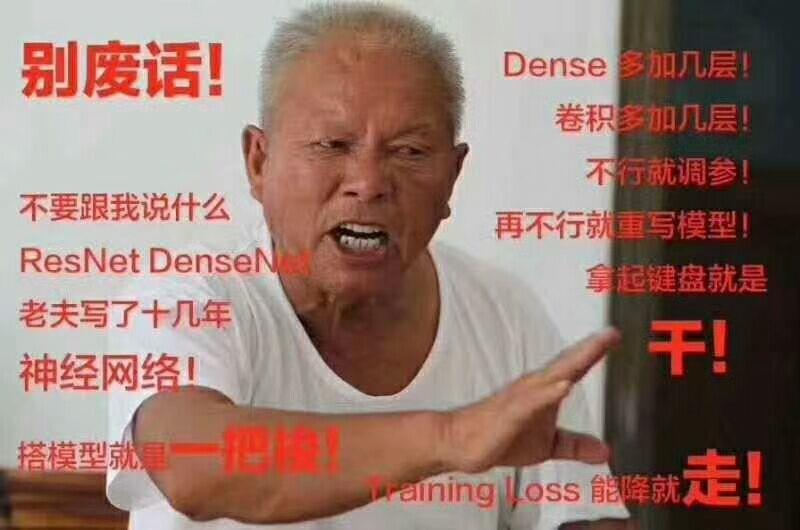展开全文深度学习 机器学习 异常检测
• 自编码器及其变形很多，本篇博客目前主要基于普通自编码器、欠完备自编码器、稀疏自编码器和去噪自编码器，会提供理论+实践（有的理论本人没有完全理解，就先没有写上，后更）。另外，关于收缩自编码器、变分自编码...

### 写在前面

因为时间原因本文有些图片自己没有画，来自网络的图片我尽量注出原链接，但是有的链接已经记不得了，如果有使用到您的图片，请联系我，必注释。

自编码器及其变形很多，本篇博客目前主要基于普通自编码器、栈式自编码器、欠完备自编码器、稀疏自编码器和去噪自编码器，会提供理论+实践（有的理论本人没有完全理解，就先没有写上，后更）。另外，关于收缩自编码器、变分自编码器、CNN自编码器、RNN自编码器及其自编码器的应用，后更。

本文展示的所有完整代码详见：完整代码
（目前只有Keras版本，有时间会写Tensorflow版本）

文章较长，PDF版点击链接：PDF版

### 一、自编码器（Autoencoder, AE）

#### 1、自编码器的结构和思想

自编码器是一种无监督的数据维度压缩和数据特征表达方法。
自编码器是神经网络的一种，经过训练后能尝试将输入复制到输出。自编码器由编码器和解码器组成，如下图所示（图片来源：深度学习浅层理解（三）— 常用模型之自编码器）：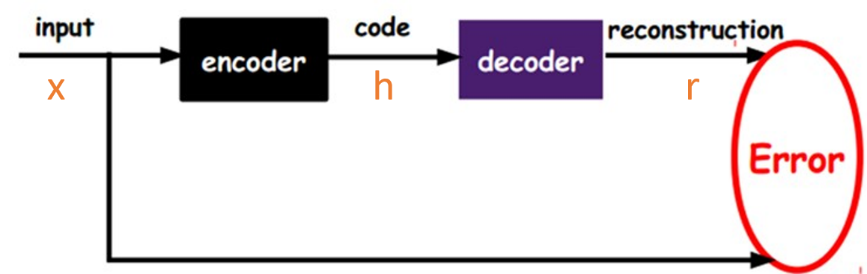h = f ( x ) h=f(x) 表示编码器， r = g ( h ) = g ( f ( x ) ) r=g(h)=g(f(x)) 表示解码器，自编码的目标便是优化损失函数 L ( x , g ( f ( x ) ) L(x,g(f(x)) ，也就是减小图中的Error。

#### 2、自编码器和前馈神经网络的比较

二者的区别和联系如下：

（1）自编码器是前馈神经网络的一种，最开始主要用于数据的降维以及特征的抽取，随着技术的不断发展，现在也被用于生成模型中，可用来生成图片等。
（2）前馈神经网络是有监督学习，其需要大量的标注数据。自编码器是无监督学习，数据不需要标注因此较容易收集。
（3）前馈神经网络在训练时主要关注的是输出层的数据以及错误率，而自编码的应用可能更多的关注中间隐层的结果。

#### 3、自编码器和受限玻尔兹曼机的比较

关于受限玻尔兹曼机的博客请参见：[受限玻尔兹曼机] 原理、求解、深度信念网络
二者的区别和联系如下：

（1）自编码器和受限自编码器的相同点如下：①都起到了降维的作用；②都可以用来对神经网络进行预训练；③训练都是无监督的。
（2）自编码器和受限自编码器的不同点如下：①自编码器希望通过非线性变换找到输入数据的特征表示，其重构的是输入分布与reconstruct分布的KL距离，它是某种确定论性的模型；而RBM则是围绕概率分布进行的，它通过输入数据的概率分布来提取中间层表示，它是某种概率论性的模型。②AE使用的是BP算法进行优化，而RBM是基于概率模型，使用CD算法进行优化。

#### 4、普通自编码器存在的问题

在普通的自编码器中，输入和输出是完全相同的，因此输出对我们来说没有什么应用价值，所以我们希望利用中间隐层的结果，比如，可以将其作为特征提取的结果、利用中间隐层获取最有用的特性等。
但是如果只使用普通的自编码器会面临什么问题呢？比如，输入层和输出层的维度都是5，中间隐层的维度也是5，那么我们使用相同的输入和输出来不断优化隐层参数，最终得到的参数可能是这样： x 1 − &gt; a 1 ， x 2 − &gt; a 2 ， … x1-&gt;a1，x2-&gt;a2，… 的参数为1，其余参数为0，也就是说，中间隐层的参数只是完全将输入记忆下来，并在输出时将其记忆的内容完全输出即可，神经网络在做恒等映射，产生数据过拟合。如下图所示（图片来源：Introduction to autoencoders.）：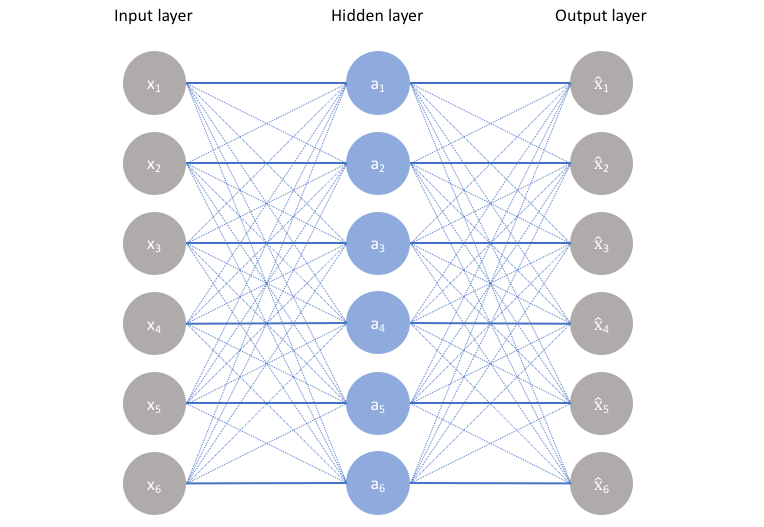上图是隐层单元数等于输入维度的情况，当然，如果是隐层单元数大于输入维度，也会发生类似的情况，即当隐层单元数大于等于输入维度时，网络可以采用完全记忆的方式，虽然这种方式在训练时精度很高，但是复制的输出对我们来说毫无意义
因此，我们会给隐层加一些约束，如限制隐藏单元数、添加正则化等，后面后介绍。

#### 5、自编码器实现与结果分析

（1）实现框架： Keras
（2）数据集： Mnist手写数字识别
（3）关键代码：

def train(x_train):
"""
build autoencoder.
:param x_train:  the train data
:return: encoder and decoder
"""
# input placeholder
input_image = Input(shape=(ENCODING_DIM_INPUT, ))

# encoding layer
hidden_layer = Dense(ENCODING_DIM_OUTPUT, activation='relu')(input_image)
# decoding layer
decode_output = Dense(ENCODING_DIM_INPUT, activation='relu')(hidden_layer)

# build autoencoder, encoder, decoder
autoencoder = Model(inputs=input_image, outputs=decode_output)
encoder = Model(inputs=input_image, outputs=hidden_layer)

# compile autoencoder

# training
autoencoder.fit(x_train, x_train, epochs=EPOCHS, batch_size=BATCH_SIZE, shuffle=True)

return encoder, autoencoder


（4）代码分析：
Keras封装的比较厉害，所以傻瓜式编程，这里是最简单的自编码器，其输入维度是28*28=784，中间单隐层的维度是2，使用的激活函数是Relu，返回encoder和autoencoder。encoder部分可以用于降维后的可视化，或者降维之后接分类等，autoencoder可以用来生成图片等（这部分代码git上都有）。结构见图如下：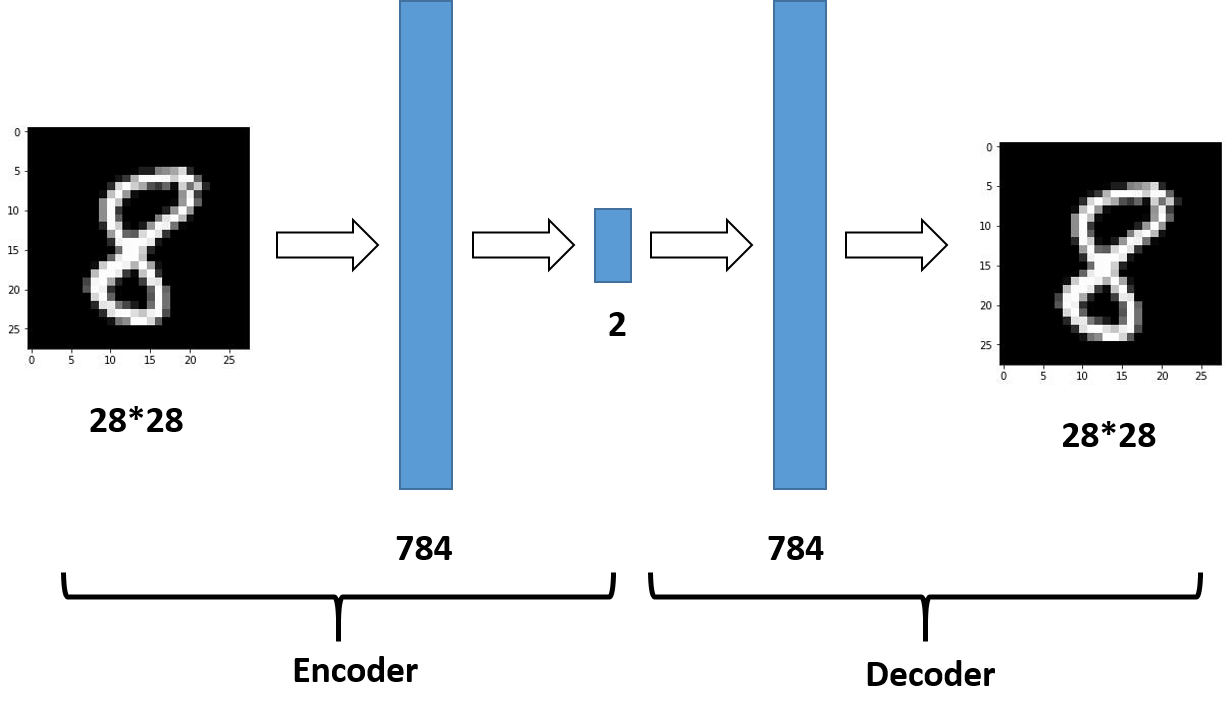（5）结果展示：

（i）Eencoder结果的可视化如图：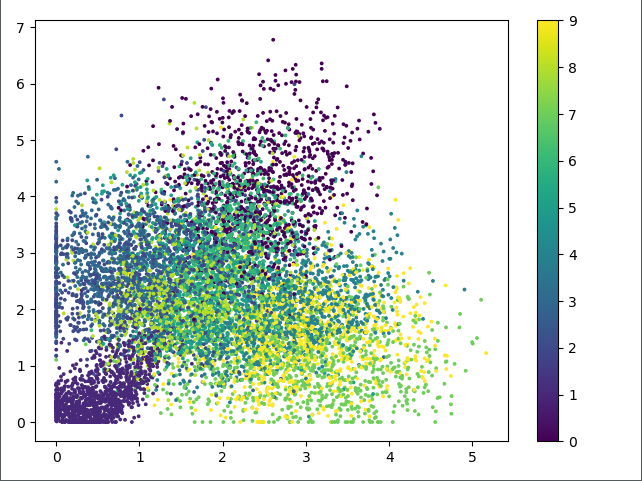上图中不同表示表示不同的数字，由图可知，自编码器降维之后的结果并不能很好地表示10个数字。

（ii）autoencoder还原之后的图片和原图片对比如下：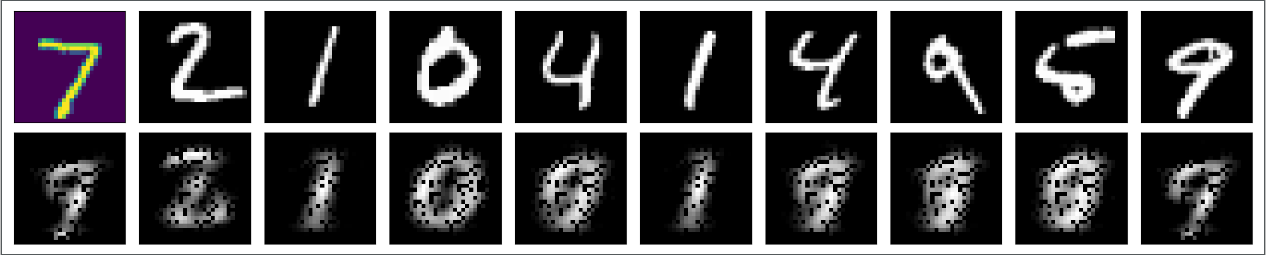上图说明，autoencoder的生成结果不是很清晰。

### 二、栈式自编码器（Stack Autoencoder）

#### 1、栈式自编码器思想

栈式自编码器又称为深度自编码器，其训练过程和深度神经网络有所区别，下面是基于栈式自编码器的分类问题的训练过程（图片来自台大李宏毅老师的PPT）：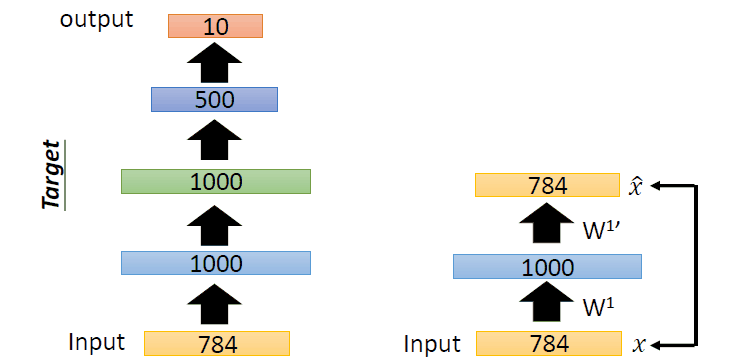即过程如下：
首先，训练784->1000->784的自编码器，而后已经固定已经训练好的参数和1000维的结果，训练第二个自编码器：1000->1000->1000，而后固定已经训练好的参数和训练的中间层结果，训练第三个自编码器：1000->500->1000，固定参数和中间隐层的结果。此时，前3层的参数已经训练完毕，此时，最后一层接一个分类器，将整体网络使用反向传播进行训练，对参数进行微调。这便是使用栈式自编码器进行分类的整体过程。
注：encoder和decoder的参数可以是对称的，也可以是非对称的。

#### 2、栈式自编码器的特点

①增加隐层可以学到更复杂的编码，每一层可以学习到不同的信息维度。
②若层数太深，encoder过于强大，可以将学习将输入映射为任意数（然后decoder学习其逆映射）。这一编码器可以很好的重建数据，但它并没有在这一过程中学到有用的数据表示。

#### 3、栈式自编码器和深度信念网络的异同点

①自编码器栈式自编码器；受限玻尔兹曼机深度信念网络
②栈式自编码器和深度信念网络都是逐层训练。
③栈式自编码器和深度信念网络的训练方法不同，栈式自编码器使用BP算法训练参数，深度信念网络使用的是对比散度算法。

#### 4、栈式自编码器实现与结果分析

（1）实现框架： Keras
（2）数据集： Mnist手写数字识别
（3）关键代码：

def train(x_train):

# input placeholder
input_image = Input(shape=(ENCODING_DIM_INPUT, ))

# encoding layer
encode_layer1 = Dense(ENCODING_DIM_LAYER1, activation='relu')(input_image)
encode_layer2 = Dense(ENCODING_DIM_LAYER2, activation='relu')(encode_layer1)
encode_layer3 = Dense(ENCODING_DIM_LAYER3, activation='relu')(encode_layer2)
encode_output = Dense(ENCODING_DIM_OUTPUT)(encode_layer3)

# decoding layer
decode_layer1 = Dense(ENCODING_DIM_LAYER3, activation='relu')(encode_output)
decode_layer2 = Dense(ENCODING_DIM_LAYER2, activation='relu')(decode_layer1)
decode_layer3 = Dense(ENCODING_DIM_LAYER1, activation='relu')(decode_layer2)
decode_output = Dense(ENCODING_DIM_INPUT, activation='tanh')(decode_layer3)

# build autoencoder, encoder
autoencoder = Model(inputs=input_image, outputs=decode_output)
encoder = Model(inputs=input_image, outputs=encode_output)

# compile autoencoder

# training
autoencoder.fit(x_train, x_train, epochs=EPOCHS, batch_size=BATCH_SIZE, shuffle=True)

return encoder, autoencoder


栈式自编码器相当于深度网络的过程，主要注意维度对应即可，另外，这里设置的encoder和decoder的维度是对称的。其架构图如下：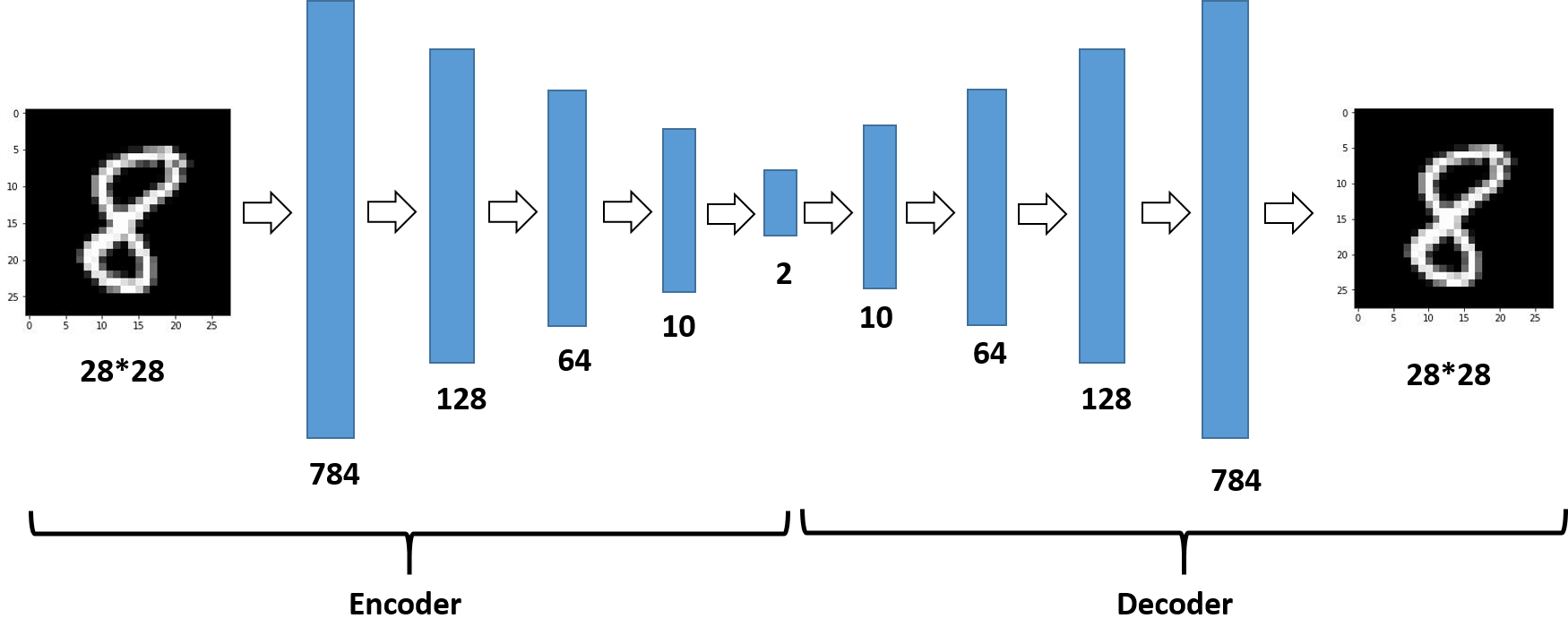（4）结果展示：

（i）Eencoder结果的可视化如图：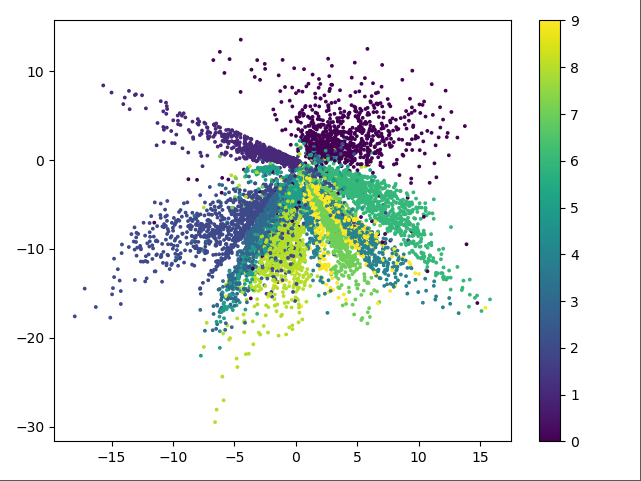上图中不同表示表示不同的数字，由图可知，栈式自编码器的效果相比较普通自编码器好很多，这里基本能将10个分类全部分开。

（ii）autoencoder还原之后的图片和原图片对比如下：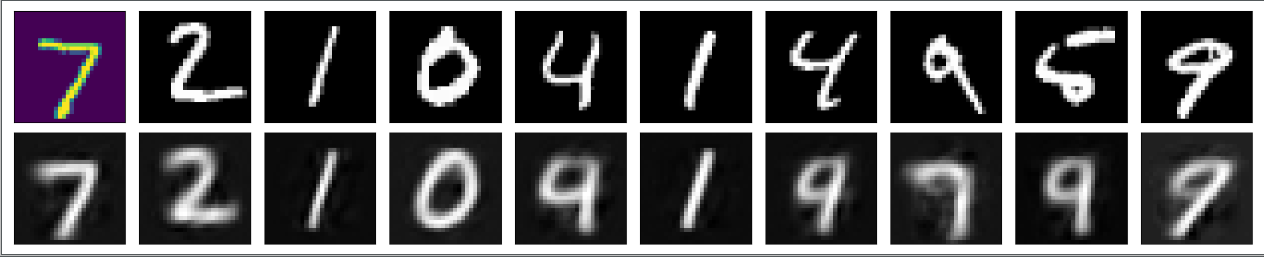### 三、欠完备自编码器（Undercomplete Autoencoder）

#### 1、欠完备自编码器的思想

由上述自编码器的原理可知，当隐层单元数大于等于输入维度时，网络会发生完全记忆的情况，为了避免这种情况，我们限制隐层的维度一定要比输入维度小，这就是欠完备自编码器，如下图所示（图片来源：Introduction to autoencoders.）。 学习欠完备的表示将强制自编码器捕捉训练数据中最显著的特征。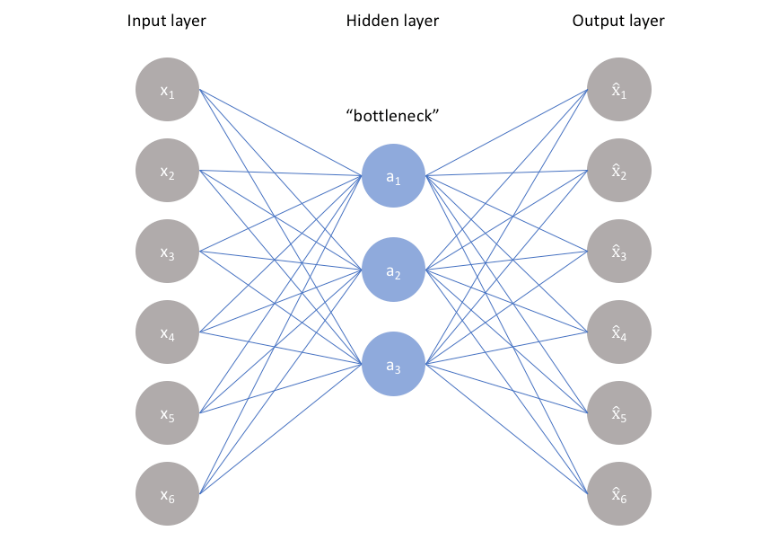#### 2、欠完备自编码器和主成分分析（PCA）的比较

实际上，若同时满足下列条件，欠完备自编码器的网络等同于PCA，其会学习出于PCA相同的生成子空间：

• 每两层之间的变换均为线性变换。
• 目标函数 L ( x , g ( f ( x ) ) L(x,g(f(x)) 为均方误差。

因此，拥有非线性编码器函数 f f 和非线性解码器函数 g g 的自编码器能够学习出比PCA更强大的知识，其是PCA的非线性推广。下图是在二维空间中PCA算法和自编码器同时作用在二维点上做映射的结果（图片来源：Introduction to autoencoders.） ，从图中可以看出，自编码器具有更好的表达能力，其可以映射到非线性函数。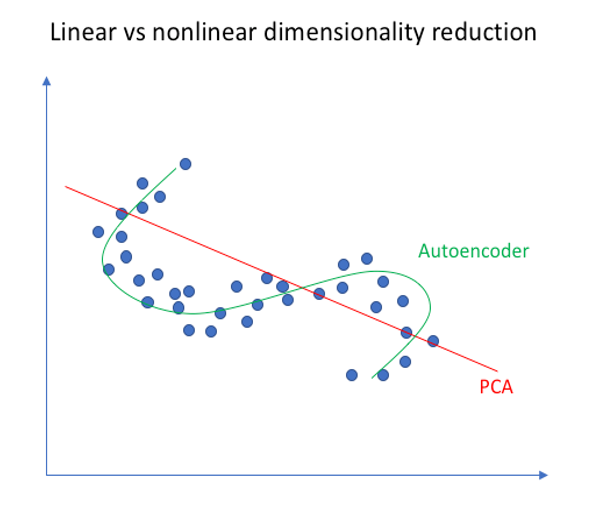#### 3、欠完备自编码器特点

• 防止过拟合，并且因为隐层编码维数小于输入维数，可以学习数据分布中最显著的特征。
• 若中间隐层单元数特别少，则其表达信息有限，会导致重构过程比较困难。

### 四、稀疏自编码器（Sparse Autoencoder）

#### 1、稀疏自编码器思想

稀疏自编码器是加入正则化的自编码器，其未限制网络接收数据的能力，即不限制隐藏层的单元数。
所谓稀疏性限制是指：

若激活函数是sigmoid，则当神经元的输出接近于1的时候认为神经元被激活，输出接近于0的时候认为神经元被抑制。使得大部分神经元别抑制的限制叫做稀疏性限制。若激活函数是tanh，则当神经元的输出接近于-1的时候认为神经元是被抑制的。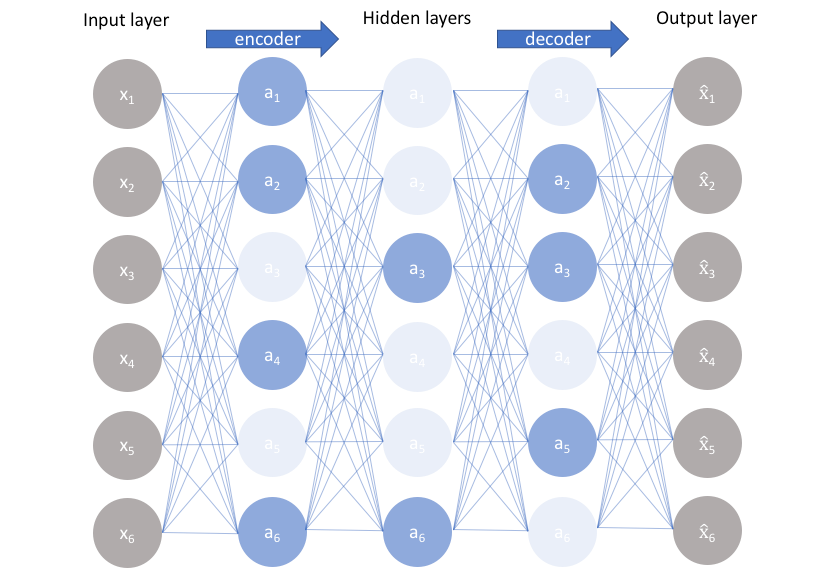如上图所示（图片来源：Introduction to autoencoders. ），浅色的神经元表示被抑制的神经元，深色的神经元表示被激活的神经元。通过稀疏自编码器，我们没有限制隐藏层的单元数，但是防止了网络过度记忆的情况。
稀疏自编码器损失函数的基本表示形式如下：
L s p a r s e ( x , g ( f ( x ) ) ) = L ( x , g ( f ( x ) ) ) + Ω ( h ) L_{sparse}(x, g(f(x)))=L(x, g(f(x))) + \Omega (h)
其中 g ( h ) g(h) 是解码器的输出，通常 h h 是编码器的输出，即 h = f ( x ) h=f(x)

#### 2、损失函数和BP函数推导

损失函数可以加入L1正则化，也可以加入KL散度，下面是对加入KL散度的损失函数的分析。
损失函数的分析如下：
假设 a j ( 2 ) ( x ) a_j^{(2)}(x) 表示在给定输入 x x 的情况下，自编码网络隐层神经元 j j 的激活度，则神经元在所有训练样本上的平均激活度为：
ρ j ^ = 1 m ∑ i = 1 m [ a j ( 2 ) ( x i ) ] \hat{\rho_j}=\frac{1}{m}\sum_{i=1}^{m}[a_j^{(2)}(x^{i})]
其中， a j ( 2 ) = f ( w j ( 1 ) x ( i ) + b j ( 1 ) ) a_j^{(2)}=f(w_j^{(1)}x^{(i)}+b_j^{(1)}) ，我们的目的使得网络激活神经元稀疏，所以可以引入一个稀疏性参数 ρ \rho ，通常 ρ \rho 是一个接近于0的值（即表示隐藏神经元中激活神经元的占比）。则若可以使得 ρ j ^ = ρ \hat{\rho_j}=\rho ，则神经元在所有训练样本上的平均激活度 ρ j ^ \hat{\rho_j} 便是稀疏的，这就是我们的目标。为了使得 ρ j ^ = ρ \hat{\rho_j}=\rho ，我们使用KL散度衡量二者的距离，两者相差越大，KL散度的值越大，KL散度的公式如下：
∑ j = 1 s 2 K L ( ρ ∣ ∣ ρ j ^ ) = ∑ j = 1 s 2 [ ρ log ⁡ ρ ρ j ^ + ( 1 − ρ ) log ⁡ 1 − ρ 1 − ρ j ^ ] \sum_{j=1}^{s_2}KL(\rho||\hat{\rho_j})=\sum_{j=1}^{s_2} [\rho \log \frac{\rho}{\hat{\rho_j}} + (1-\rho) \log \frac{1-\rho}{1-\hat{\rho_j}}]
ρ \rho 表示平均激活度的目标值。因此损失函数如下：
J s p a r s e ( W , b ) = J ( W , b ) + β ∑ j = 1 s 2 K L ( ρ ∣ ∣ ρ j ^ ) J_{sparse}(W,b)=J(W,b)+\beta \sum_{j=1}^{s_2}KL(\rho||\hat{\rho_j})
其中 J ( W , b ) J(W, b) 便是NN网络中的普通的代价函数，可以使用均方误差等。

反向传播的分析如下：
上式代价函数，左部分就是之前BP的结果，结果如下：
∂ J ( W , b ) ∂ z i ( 2 ) = ∑ j = 1 s 2 W j i ( 2 ) ∂ J ( W , b ) ∂ z i ( 3 ) f ′ ( z i ( 2 ) ) \frac{\partial J(W,b)}{ \partial z_i^{(2)}} = \sum_{j=1}^{s_2}W_{ji}^{(2)}\frac{\partial J(W,b)}{ \partial z_i^{(3)}}{f}&#x27;(z_i^{(2)})
可参考反向传导算法

右部分的求导如下：

∂ ∑ j = 1 s 2 K L ( ρ ∣ ∣ ρ j ^ ) ∂ z i ( 2 ) = ∂ K L ( ρ ∣ ∣ ρ i ^ ) ∂ z i ( 2 ) = ∂ K L ( ρ ∣ ∣ ρ i ^ ) ∂ ρ i ^ ⋅ ∂ ρ i ^ ∂ z i ( 2 ) = ∂ ( ρ log ⁡ ρ ρ i ^ + ( 1 − ρ ) log ⁡ 1 − ρ 1 − ρ i ^ ) ∂ ρ i ^ ⋅ ∂ ρ i ^ ∂ z i ( 2 ) = ( − ρ ρ i ^ + 1 − ρ 1 − ρ i ^ ) ⋅ f ′ ( z i ( 2 ) ) \begin{aligned} \frac{\partial \sum_{j=1}^{s_2}KL(\rho||\hat{\rho_j})}{\partial z_i^{(2)}} &amp;= \frac{\partial KL(\rho||\hat{\rho_i})}{\partial z_i^{(2)}} \newline \\ &amp;= \frac{\partial KL(\rho||\hat{\rho_i})}{\partial \hat{\rho_i}}\cdot \frac{\partial \hat{\rho_i}}{\partial z_i^{(2)}} \\ &amp;= \frac{\partial(\rho \log \frac{\rho}{\hat{\rho_i}}+(1-\rho) \log \frac{1-\rho}{1-\hat{\rho_i}})}{\partial\hat{\rho_i}}\cdot \frac{\partial \hat{\rho_i}}{\partial z_i^{(2)}} \\ &amp;= (-\frac{\rho}{\hat{\rho_i}} + \frac{1-\rho}{1-\hat{\rho_i}}) \cdot {f}&#x27;(z_i^{(2)}) \end{aligned}
因此 J s p a r s e ( W , b ) J_{sparse}(W,b) 的求导结果如下：
∂ J s p a r s e ( W , b ) ∂ z i ( 2 ) = ( ( ∑ j = 1 s 2 W j i ( 2 ) ∂ J ( W , b ) ∂ z i ( 3 ) ) + β ( − ρ ρ i ^ + 1 − ρ 1 − ρ i ^ ) ) f ′ ( z i ( 2 ) ) \frac{\partial J_{sparse}(W,b)}{ \partial z_i^{(2)}}= ((\sum_{j=1}^{s_2}W_{ji}^{(2)}\frac{\partial J(W,b)}{ \partial z_i^{(3)}})+ \beta(-\frac{\rho}{\hat{\rho_i}} + \frac{1-\rho}{1-\hat{\rho_i}})){f}&#x27;(z_i^{(2)})
此即反向传播的方程，根据此方程可以参数 W W b b 进行更新。

#### 3、Mini-Batch的情况

平均激活度是根据所有样本计算出来的，所以在计算任何单元的平均激活度之前，需要对所有样本计算一下正向传播，从而获得平均激活度，所以使用小批量时计算效率很低。要解决这个问题，可采取的方法是只计算Mini-Batch中包含的训练样本的平均激活度，然后在Mini-Batch之间计算加权值
ρ ^ j t = λ ρ ^ j t − 1 + ( 1 − λ ) ρ ^ j t \hat{\rho}_j^t=\lambda\hat{\rho}_j^{t-1}+(1-\lambda)\hat{\rho}_j^t
其中， ρ ^ j t \hat{\rho}_j^t 是时刻 t t 的Mini-Batch的平均激活度， ρ ^ j t − 1 \hat{\rho}_j^{t-1} 是时刻t-1的Mini-Batch的平均激活度。若\lambda大，则时刻 t − 1 t-1 的Mini-Batch的平均激活度所占比重大，否则，时刻t的Mini-Batch的平均激活度所占比重大。

#### 4、稀疏限制和L1/L2正则化的关系

①稀疏限制是对激活函数的结果增加限制，使得尽量多的激活函数的结果为0（如果激活函数是tanh，则为-1）
②L2/L1是对参数增加限制，使得尽可能多的参数为0。
若自编码器编码函数 f ( w x + b ) f(wx+b) ，若 f f 是一个线性函数，则编码器便可以写成 w x + b wx+b ，限制激活函数的结果尽量为0，即是限制 w w 尽量为0，此时稀疏限制和正则化限制相同。

#### 5、稀疏自编码器在分类中的应用

稀疏自编码器一般用来学习特征，以便用于像分类这样的任务。如下图（图片来源：为什么稀疏自编码器很少见到多层的？）：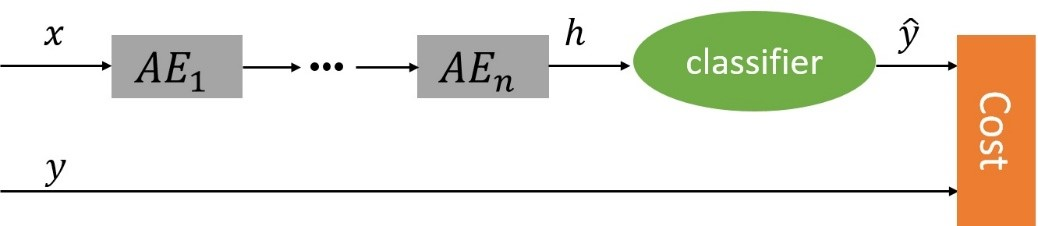上述过程不是一次训练的，可以看到上面只有编码器没有解码器，因此其训练过程是自编码器先使用数据训练参数，然后保留编码器，将解码器删除并在后面接一个分类器，并使用损失函数来训练参数已达到最后效果。

#### 5、稀疏编码器实现与结果分析

（1）框架： Keras
（2）数据集： Mnist手写数字识别
（3）关键代码：

def train(x_train):

# input placeholder
input_image = Input(shape=(ENCODING_DIM_INPUT, ))

# encoding layer
# *****!!! this code is changed compared with Autoencoder, adding the activity_regularizer to make the input sparse.
encode_layer1 = Dense(ENCODING_DIM_LAYER1, activation='relu', activity_regularizer=regularizers.l1(10e-6))(input_image)
# *******************************************************
encode_layer2 = Dense(ENCODING_DIM_LAYER2, activation='relu')(encode_layer1)
encode_layer3 = Dense(ENCODING_DIM_LAYER3, activation='relu')(encode_layer2)
encode_output = Dense(ENCODING_DIM_OUTPUT)(encode_layer3)

# decoding layer
decode_layer1 = Dense(ENCODING_DIM_LAYER3, activation='relu')(encode_output)
decode_layer2 = Dense(ENCODING_DIM_LAYER2, activation='relu')(decode_layer1)
decode_layer3 = Dense(ENCODING_DIM_LAYER1, activation='relu')(decode_layer2)
decode_output = Dense(ENCODING_DIM_INPUT, activation='tanh')(decode_layer3)

# build autoencoder, encoder
autoencoder = Model(inputs=input_image, outputs=decode_output)
encoder = Model(inputs=input_image, outputs=encode_output)

# compile autoencoder

# training
autoencoder.fit(x_train, x_train, epochs=EPOCHS, batch_size=BATCH_SIZE, shuffle=True)

return encoder, autoencoder


这里是以多层的自编码器举例，单隐层的同样适用，主要是在第一层加一个正则化项，activity_regularizer=regularizers.l1(10e-6)说明加入的是L1正则化项，10e-6是正则化项系数，完整代码可参见最开始的git。其架构如下：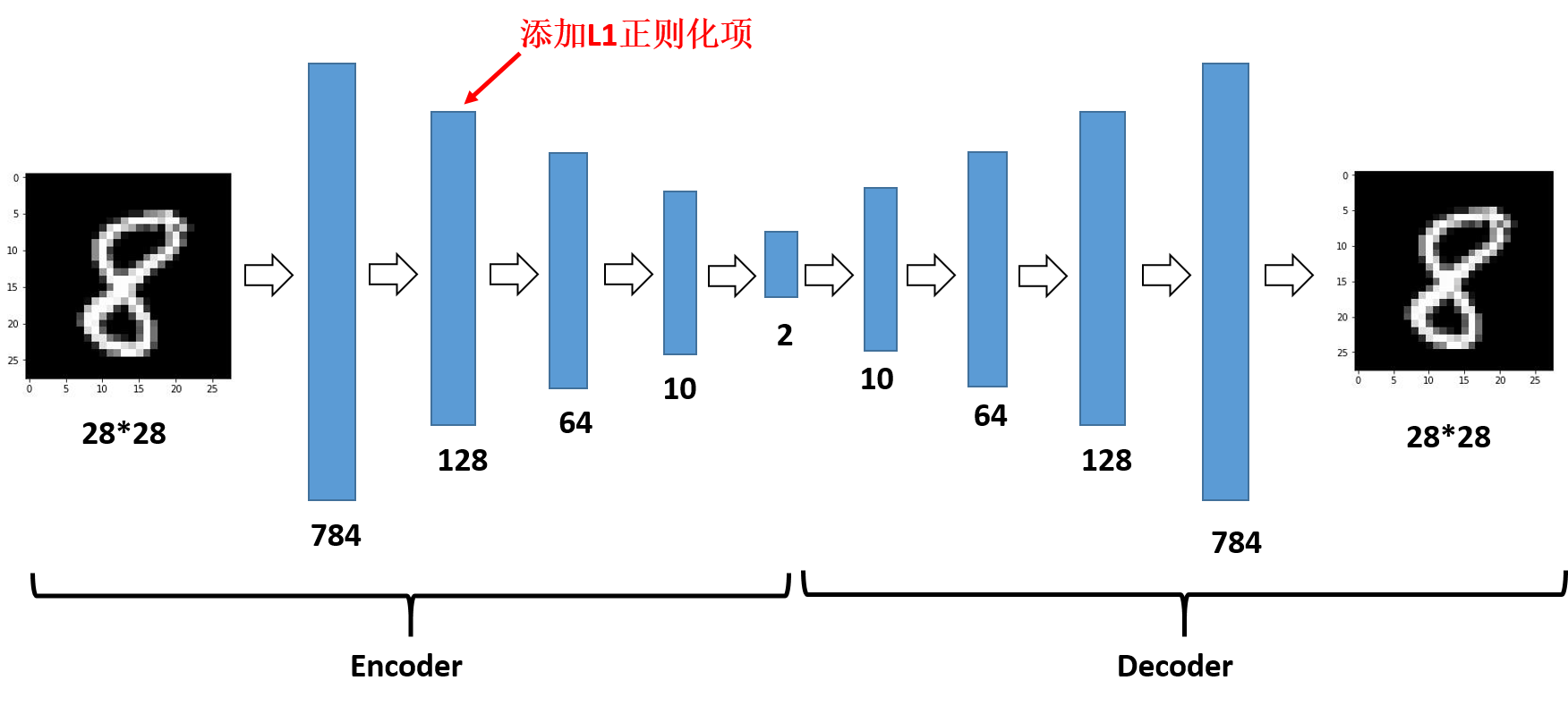（4）结果展示：
（i）Encoder结果的可视化如图：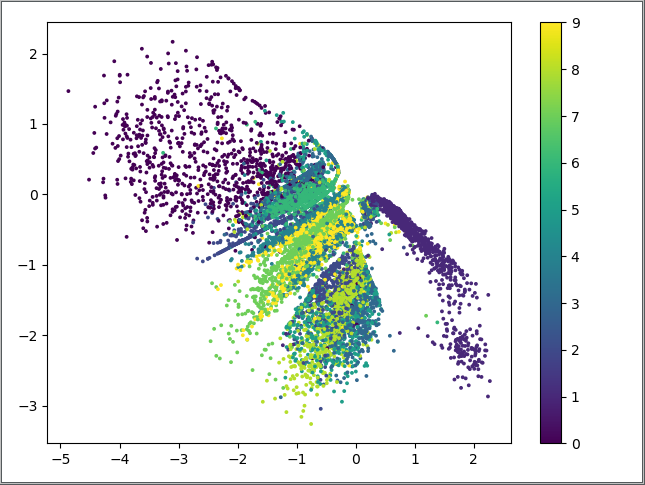上图中不同颜色表示不同的数字，由图可知，这个编码器的分类效果还可以，比自编码器好很多，但是看起来还是作用不大，因为大部分作用需要归功于栈式自编码器。

（ii）autoencoder还原之后的图片和原图片对比如下：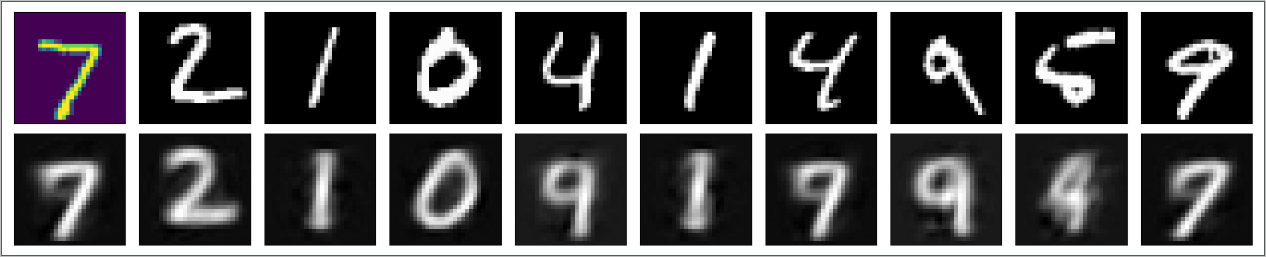### 五、去噪自编码器（Denoising Autoencoder）

#### 1、去噪自编码器思想

去噪自编码器是一类接受损失数据作为输入，并训练来预测原始未被损坏的数据作为输出的自编码器。 如下图所示（图片来自花书）：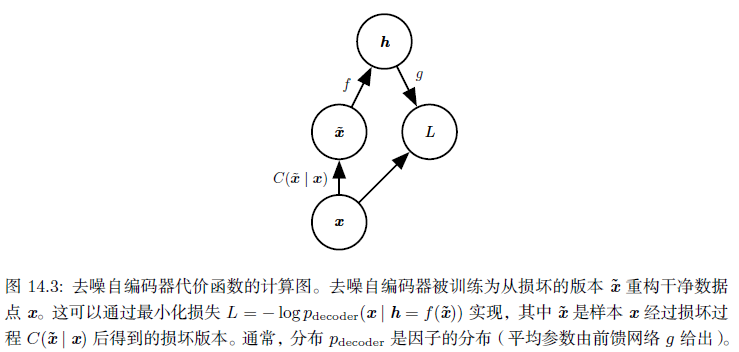其训练过程如下：
引入一个损坏过程 C ( x ~ ∣ x ) C(\tilde{x}|x) ，这个条件分布代表给定数据样本 x x 产生损坏样本 x ~ \tilde{x} 的概率。自编码器学习重构分布 p r e c o n s t r u c t ( x ∣ x ~ ) p_{reconstruct}(x|\tilde{x}) :

• 从训练数据中采一个训练样本 x x
• C ( x ~ ∣ X = x ) C(\tilde{x}|X=x) 采一个损坏样本 x ~ \tilde{x}
• ( x ~ , x ) (\tilde{x}, x) 作为训练样本来估计自编码器的重构分布 p r e c o n s t r u c t ( x ∣ x ~ ) = p d e c o d e r ( x ∣ h ) p_{reconstruct}(x|\tilde{x})=p_{decoder}(x|h) ，其中 h h 是编码器 f ( x ~ ) f(\tilde{x}) 的输出， p d e c o d e r p_{decoder} 根据解码函数 g ( h ) g(h) 定义。

去噪自编码器中作者给出的直观解释是：和人体感官系统类似，比如人的眼睛看物体时，如果物体的某一小部分被遮住了，人依然能够将其识别出来，所以去噪自编码器就是破坏输入后，使得算法学习到的参数仍然可以还原图片。
注： 噪声可以是添加到输入的纯高斯噪声，也可以是随机丢弃输入层的某个特性。

#### 2、去噪自编码器和Dropout

噪声可以是添加到输入的纯高斯噪声，也可以是随机丢弃输入层的某个特性，即如果 C ( x ~ ∣ X = x ) C(\tilde{x}|X=x) 是一个二项分布，则其表现为下图所示内容，即经过处理的 x x 的结果是保留或者舍掉，也就是说， C ( x ~ ∣ X = x ) C(\tilde{x}|X=x) 会舍去一部分内容，保留一部分内容：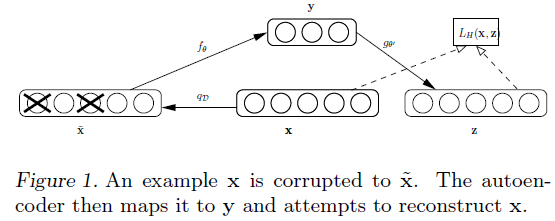这个思想类似于Dropout，但是二者还有一些区别：

（1） 去噪自编码器操作的是输入数据，相当于对输入数据去掉一部分内容；而Dropout操作的是网络隐藏层，相当于去掉隐藏层的一部分单元。
（2） Dropout在分层预训练权值的过程中是不参与的，只是后面的微调部分会加入；而去噪自编码器是在每层预训练的过程中作为输入层被引入，在进行微调时不参与

#### 3、去噪自编码器和PCA

去噪自编码器来源于论文[Vincent P, Larochelle H, Bengio Y, et al. Extracting and composing robust features with denoising autoencoders[C]//Proceedings of the 25th international conference on Machine learning. ACM, 2008: 1096-1103.]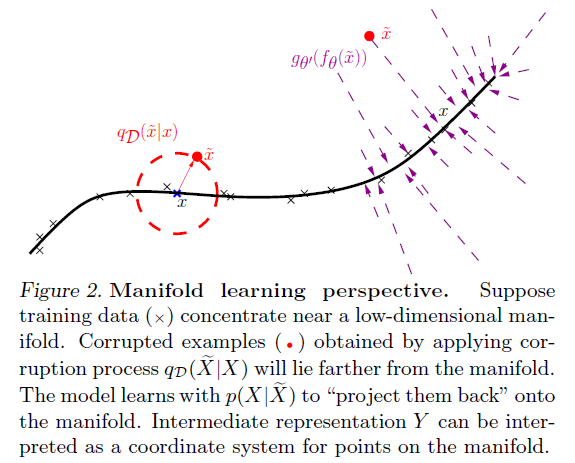上图是去噪自编码器从流形角度的原理，图中黑色的曲线表示原始的局部流型，我们通过 C ( x ~ ∣ X = x ) C(\tilde{x}|X=x) 将其映射到一个圆的某一点， x ~ \tilde{x} 表示添加噪声之后数据点。我们的目标是使得添加噪声之后的点能够映射到原始点，这样损失值为最小，即：图中红色箭头是噪声添加的向量场，而紫色部分是重构过程中需要不断查找的向量场。
因此，可以理解为，去噪自编码器的局部就是简化的PCA原理，其是对PCA的非线性扩展。
除此之外，文章还从信息论文、随机算子等角度理论上证明了去噪自编码器的可行性，有兴趣的读者可以参考上面提到的论文。

#### 4、去噪自编码器效果

论文中的实验基于Minst数据集，其实验结果如下: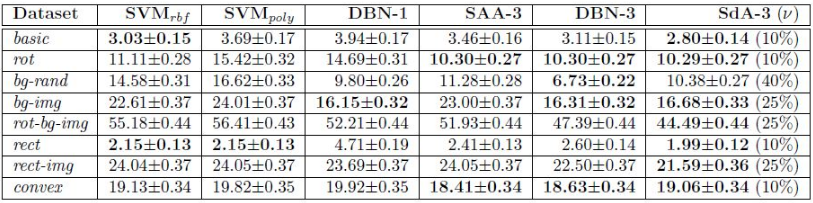其中，第一个和第二个baseline是使用高斯核和多项式核的SVM，DBN-1是1层隐层单元的深度信念网络，DBN-3是3层隐层单元的深度信念网络，SAA-3是使用栈式自编码器初始化之后的3层深度网络，最后的Sda-3是3层的栈式去噪自编码器。从表中可以看出，使用去噪自编码器的结果优于其他网络。
除此之外，网站Denoising Autoencoders (dA)有去噪自编码器的代码和实验，其去噪前和去噪后过滤器的对比结果如下：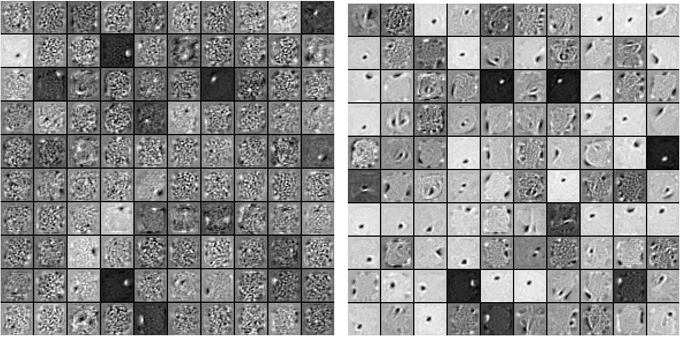左图是去噪之前的过滤器数据，右图是去噪之后的过滤器数据，从图中可以看出，去噪自编码器学习到的特征更具代表性。。

#### 5、去噪自编码器特点

• 普通的自编码器的本质是学一个相等函数，即输入和输出是同一个内容，这种相等函数的缺点便是当测试样本和训练样本不符合同一个分布时，在测试集上效果不好，而去噪自编码器可以很好地解决这个问题。
• 欠完备自编码器限制学习容量，而去噪自编码器允许学习容量很高，同时防止在编码器和解码器学习一个无用的恒等函数。
• 经过了加入噪声并进行降噪的训练过程，能够强迫网络学习到更加鲁棒的不变性特征，获得输入的更有效的表达。

#### 6、去噪编码器实现与结果分析

（1）框架： Keras
（2）数据集： Mnist手写数字识别
（3）关键代码：

def addNoise(x_train, x_test):
"""
:return:
"""
x_train_noisy = x_train + NOISE_FACTOR * np.random.normal(loc=0.0, scale=1.0, size=x_train.shape)
x_test_noisy = x_test + NOISE_FACTOR * np.random.normal(loc=0.0, scale=1.0, size=x_test.shape)

x_train_noisy = np.clip(x_train_noisy, 0., 1.)     # limit into [0, 1]
x_test_noisy = np.clip(x_test_noisy, 0., 1.)   # limit into [0, 1]

return x_train_noisy, x_test_noisy


去噪自编码器主要是对输入添加噪声，所以训练过程是不需要改变的，只需要改变输入和输出。上述便是对输入添加噪声的过程，NOISE_FACTOR * np.random.normal(loc=0.0, scale=1.0, size=x_train.shape)便是添加的噪声。 np.clip()是截取函数，将数值限制在0~1之间。其架构如下：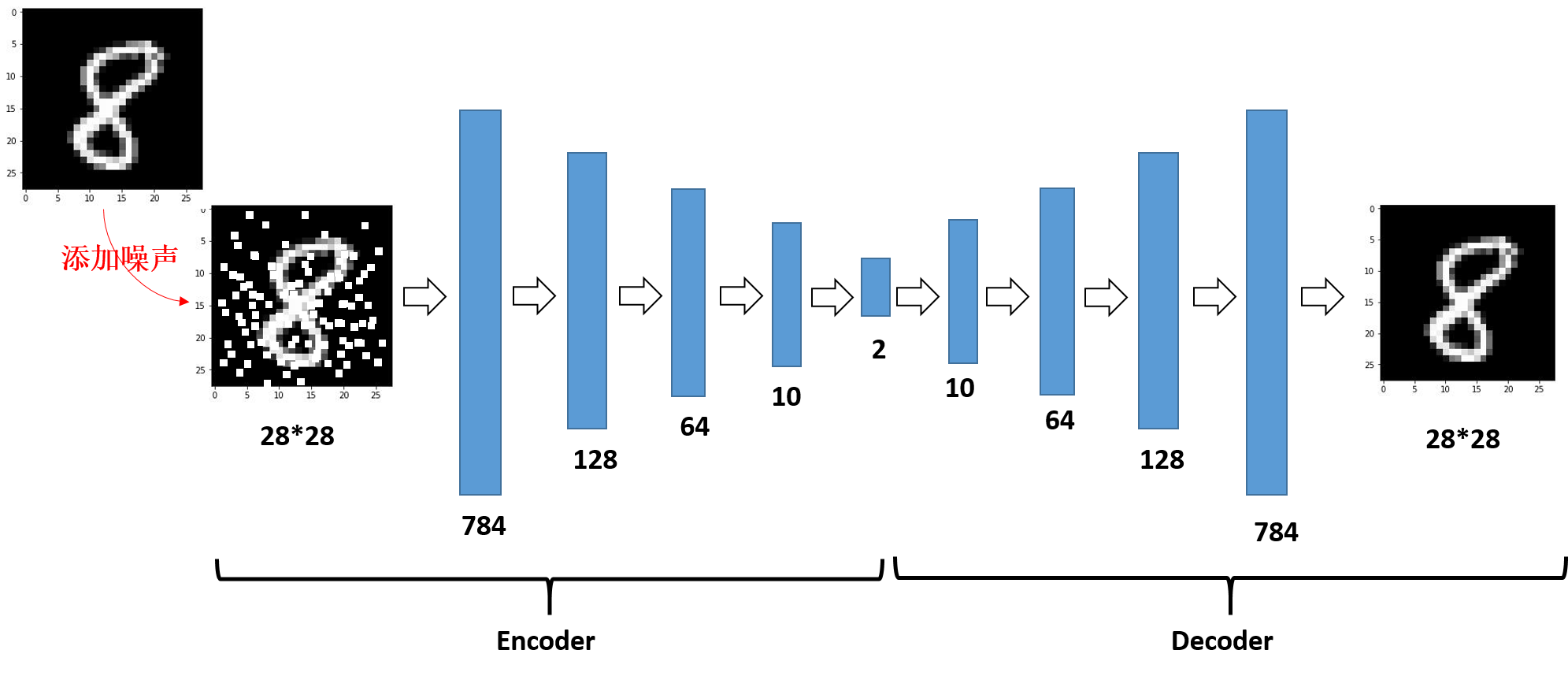（4）结果展示：
（i）Eencoder结果的可视化如图：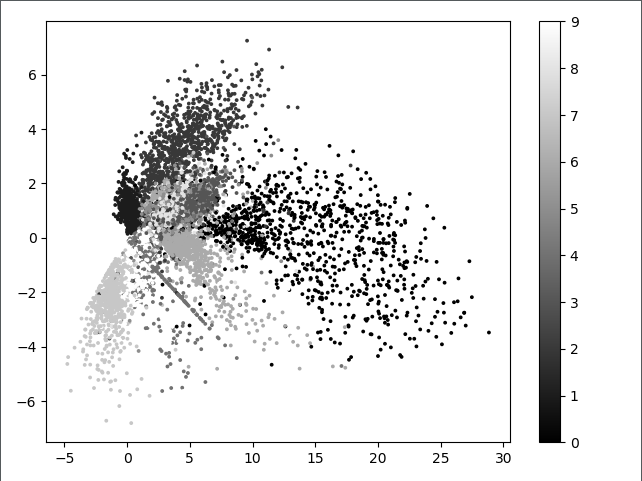上图中不同表示表示不同的数字，这里不是很直观，看下面的图片对比

（ii）autoencoder还原之后的图片和原图片对比如下：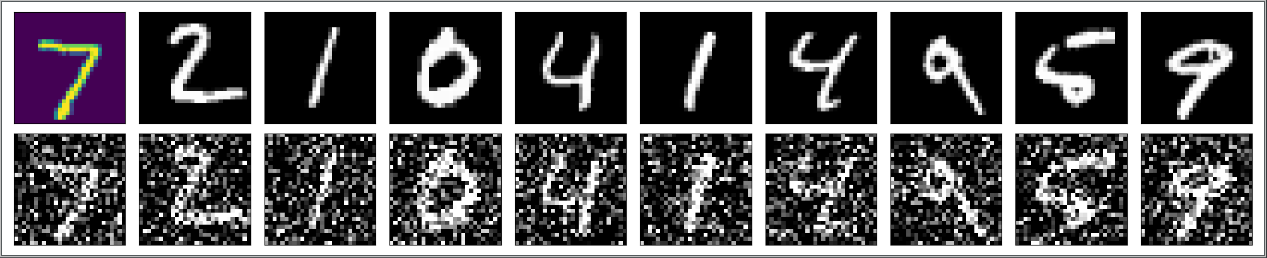上图是添加噪声的效果对比，第一行表示原数据，第二行表示噪声处理过后的数据。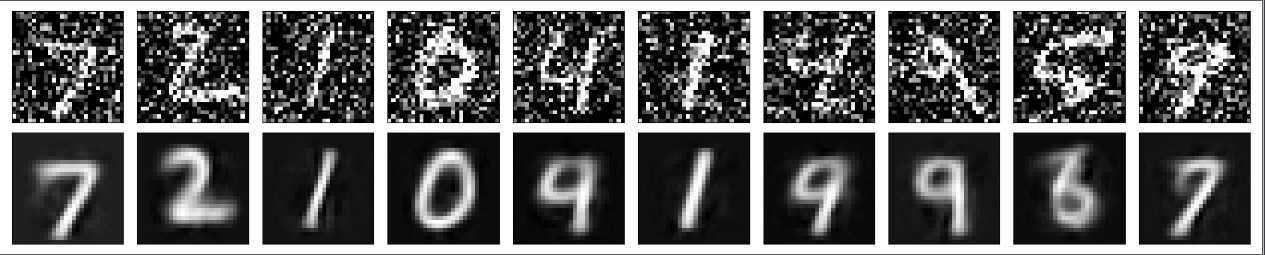上图根据噪声数据还原图片的对比，第一行表示噪声处理过后的数据，第二行表示去噪自编码器decoder还原之后的结果，上图可以看出，去噪自编码器的效果还是可以的。

### 六、卷积自编码器（convolutional Autoencoder）

#### 1、卷积自编码器思想

卷积自编码器和普通自编码器的区别在于其encoder和decoder都是卷积神经网络，相应的，encoder使用的是卷积操作和池化操作，而decoder中使用的反卷积操作和反卷积操作，关于卷积、反卷积、池化和反池化的内容详见：[CNN] 卷积、反卷积、池化、反池化

#### 2、去噪编码器实现与结果分析

（1）框架： Keras
（2）数据集： Mnist手写数字识别
（3）关键代码：

def train(x_train):
"""
build autoencoder.
:param x_train:  the train data
:return: encoder and decoder
"""
# input placeholder
input_image = Input(shape=(28, 28, 1))

# encoding layer
x = Conv2D(CHANNEL_1, (3, 3), activation='relu', padding="same")(input_image)
x = Conv2D(CHANNEL_2, (3, 3), activation='relu', padding='same')(x)

# decoding layer
x = Conv2D(CHANNEL_2, (3, 3), activation='relu', padding='same')(encoded)
x = UpSampling2D((2, 2))(x)
x = Conv2D(CHANNEL_1, (3, 3),activation='relu', padding='same')(x)
x = UpSampling2D((2, 2))(x)
decoded = Conv2D(CHANNEL_OUTPUT, (3, 3), activation='sigmoid', padding='same')(x)

# build autoencoder, encoder, decoder
autoencoder = Model(inputs=input_image, outputs=decoded)
encoder = Model(inputs=input_image, outputs=encoded)

# compile autoencoder

# training
# need return history, otherwise can not use history["acc"]
history_record = autoencoder.fit(x_train, x_train, epochs=EPOCHS, batch_size=BATCH_SIZE, shuffle=True, )

return encoder, autoencoder, history_record


注意：

i)在Keras编码中，反卷积的实现代码便是卷积操作，具体解释详见上述博客。
ii) UpSampling2D()实现的是反平均卷积的操作。

autoencoder.summary()如下: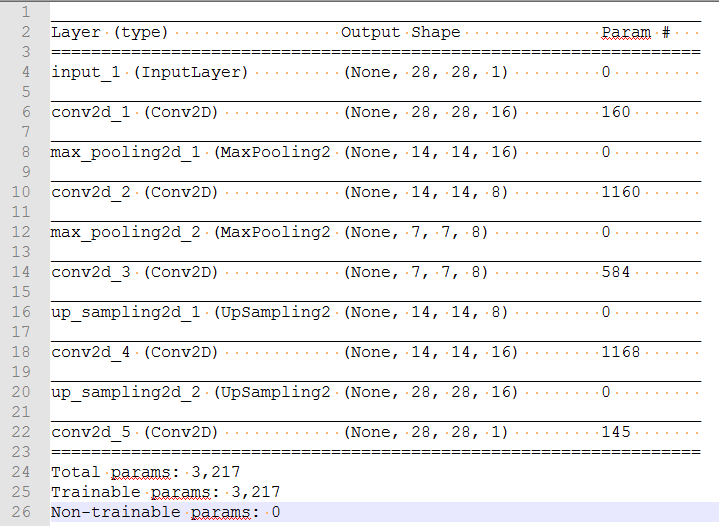代码架构图如下：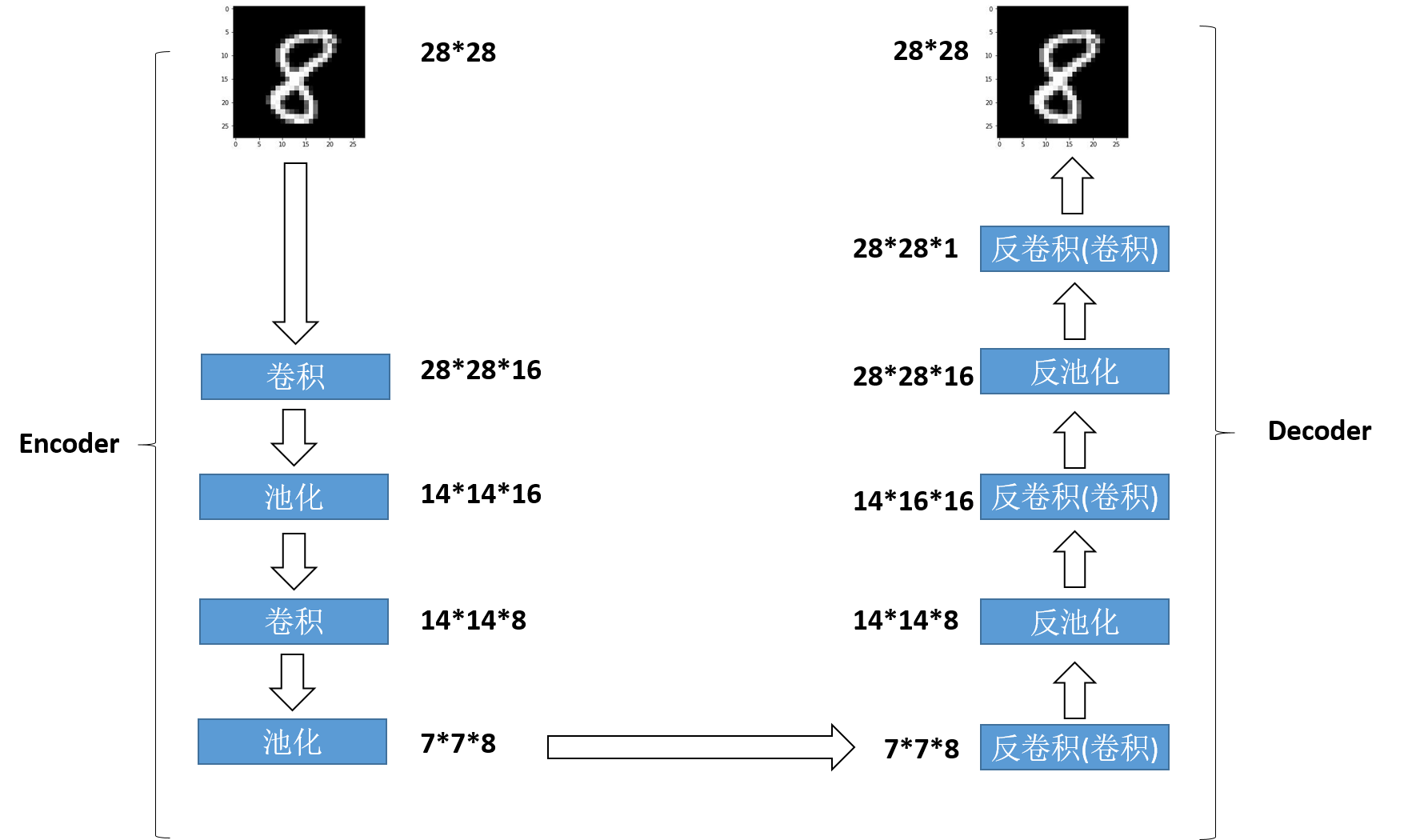（4）结果展示：
（i）autoencoder还原之后的图片和原图片对比如下：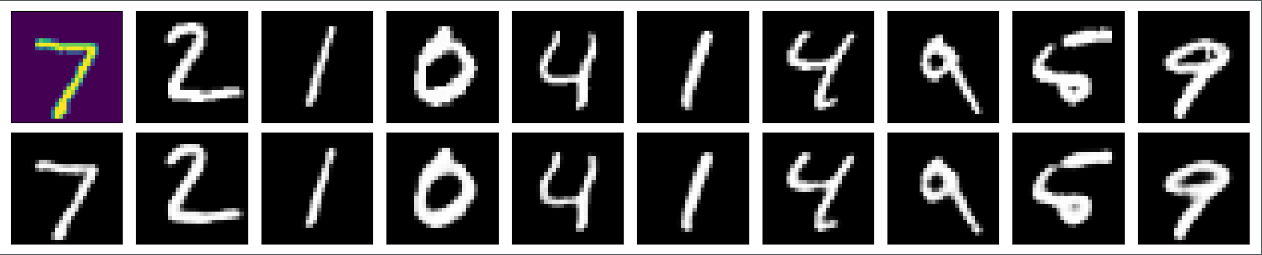上图根据原图片和生成图片的对比，第一行表示原图片，第二行表示卷积自编码器decoder还原之后的结果，上图可以看出，效果还是不错的。

（ii）loss变化图：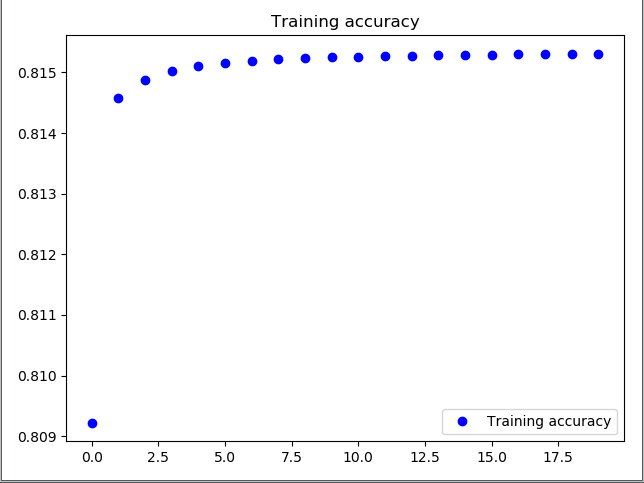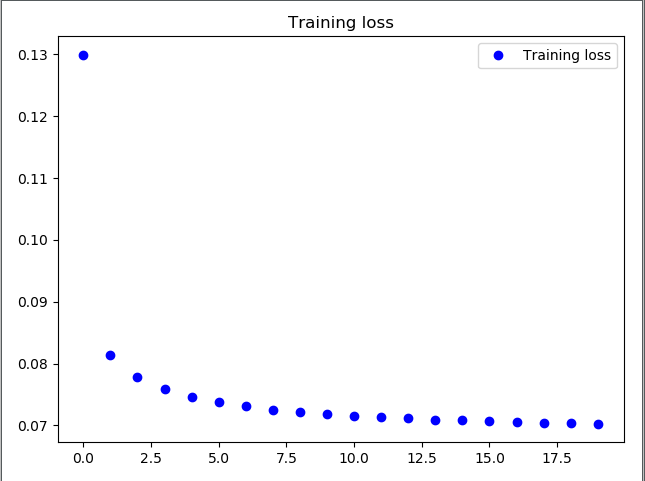由上图可以看出，虽然loss在不断降低，但是accuracy还不是非常高，一方面是和参数相关，因为性能原因，在此文章版本上对epochs设置为20，另外，网络的深度也不够，也没有加入一些其他的提高性能的小技巧。

其他参考文章：
 Kandeng. 自编码算法与稀疏性[EB/OL]. (2018/11/24)[ 2018/11/24] http://ufldl.stanford.edu/wiki/index.php/自编码算法与稀疏性
 Goodfellow I, Bengio Y, Courville A, et al. Deep learning[M]. Cambridge: MIT press, 2016.
 山下隆义. 图解深度学习[M]. 人民邮电出版社，2018：68-78
 Francois Chollet. Building Autoencoders in Keras[EB/OL]. (2018/11/24)[ 2018/11/25] https://blog.keras.io/building-autoencoders-in-keras.html

展开全文• 变分自编码器VAE：原来是这么一回事 半小时理解变分自编码器 花式解释AutoEncoder与VAE - 知乎 (zhihu.com) 【深度学习】AE与VAE_sinat_36197913的博客-CSDN博客_vae和ae 零、需要的简单数学公式 K-L散度（Kullback-...机器学习 深度学习
• 编码维数小于输入维数的欠完备自编码器可以学习数据分布最显著的特征，但如果这类自编码器的容量过大，将会专注于复制任务进而学习不到任何有益信息。 如果隐藏编码的维数与输入相等或者大于输入的过完备自编码器...人工智能 机器学习
• ## 自编码器原理概述

千次阅读 2020-01-14 16:47:58
自编码器(Auto-Encoder)，是一种利用反向传播算法使得输出值等于输入值的神经网络，它先将输入压缩成潜在空间表征，然后通过这种表征来重构输出。自编码器由两部分组成： 编码器：这部分能将输入压缩成潜在空...图像生成 计算机视觉 Keras
•     当你在看论文的时候，经常会遇到编码器、解码器、自编码器（Autoencoder）这些字眼，它们到底是干什么的呢？其主要作用又是什么呢？那么本篇主要带大家了解自编码器（Autoencoder）。 自编码器...
• 自编码器的一般结构 传统自编码器通过最小化如下目标： (公式1) 公式1中L是一个损失函数，惩罚g(f(x))与x的差异，如它们彼此差异的L2范数。若自编码器容量过大，则其将学到一个毫无意义的恒等式。 去噪自编码器...人工智能 机器学习
• ## 详解自动编码器(AE)

千次阅读 多人点赞 2019-11-21 11:57:34
降噪自编码(Denoising Auto-Encoders, DAE)(2008) 堆叠降燥自动编码器 (Stacked Denoising Auto-Encoders, SAE)(2008) 卷积自动编码器(Convolution Auto-Encoders, CAE)(2011) 变分自动编码器(Variational Auto-...Python实现
• 自编码器自编码器是用于无监督学习，高效编码的神经网络，自动编码器的目的就在于，学习一组数据的编码，通常用于数据的降维，自编码是一种无监督的算法，网络分为：输入层，隐藏层（编码层），解码层，该网络的...神经网络 深度学习
• Train Sparse Autoencoder训练稀疏自编码器 利用8个特征4177个样本的数据进行稀疏自编码器的训练，训练设置为系统默认。利用训练后的网络进行数据重建，最后给出了重建误差作为网络效果的衡量。 %% Train Sparse ...机器学习 matlab
•   在非监督学习中，最典型的一类神经网络莫过于autoencoder(自编码器)，它的目的是基于输入的unlabeled数据X={x(1),x(2),x(3),...}X={x(1),x(2),x(3),...}X=\{x^{(1)}, x^{(2)}, x^{(3)}, ...\}，通过训练得到数据...tensorflow
• 本文内容参考了Conditional VAE (Variational Auto Encoder) 条件付きVAE 是对官方网页Train Variational Autoencoder (VAE) to Generate Images的改进，该网页的翻译可见MATLAB实现自编码器（四）——变分自编码器...深度学习 matlab
• 一些特征转换技术有主成分分析（PCA）、矩阵分解、自动编码器（Autoencoders）、t-Sne、UMAP等。 本文主要介绍了主成分分析以及自动编码器两种方法，具体分析两者的优缺点，并且通过一个生动的示例进行详解。 主成分...机器学习 特征提取 pca降维
• ## 自编码器简介

千次阅读 2019-02-22 14:31:23
一、什么是自编码器（Autoencoder） 自动编码器是一种数据的压缩算法，其中数据的压缩和解压缩函数是数据相关的、有损的、从样本中自动学习的。在大部分提到自动编码器的场合，压缩和解压缩的函数是通过神经网络...
• name='decoder_output')(x) 解码器如下： decoder = Model(latent_inputs, outputs, name='decoder') decoder.summary() 整合成自编码器 自编码器 = 编码器 + 解码器 autoencoder = Model(inputs, decoder(encoder...机器学习 深度学习 数据处理 神经网络
• 【翻译： Autoencoder Feature Extraction for Classification】 【说明：Jason BrownleePhD大神...编码器压缩输入，而解码器尝试根据编码器提供的压缩版本重新创建输入。训练后，将保存编码器模型，并丢弃解码...
• ## 对抗自编码器(AAE)

千次阅读 2020-09-20 16:41:36
对抗自编码器(AAE) 自编码器转换成生成模型 通过两个目标训练：传统的重构误差函数和对抗训练函数—>将AE隐藏层向量表示的聚合后验分布与任意先验分布匹配。训练准则和VAE很像 1、编码器学到将数据分布转换...深度学习 人工智能
• 一、自编码器原理 自编码器算法属于自监督学习范畴，如果算法把x作为监督信号来学习，这里算法称为自监督学习(Self-supervised Learning) 在监督学习中神经网络的功能:。是输入的特征向量长度，是网络输出的向量...机器学习 深度学习 人工智能
• 自动编码器，主要作用： 数据去噪， 可视化降维度， 生成数据。 模型结构： 缺点：在inference时只能通过输入一个输入得到一个隐含变量Z，然后在通过Decoder生成，无法任意去构造隐含变量。 1.2 VAE计算机视觉 深度学习 自监督学习 神经网络
• 自编码器(Auto Encoder)神经网络常常用于分类，通过定义一个目标函数衡量输出与目标值之间的差异，然后通过调整系统的参数使系统尽量拟合训练数据． 而对每一层神经网络来说，前一层的输出都是可看做未加工的初始...tensorflow
• 自编码器是一种特殊的神经网络（neural network），它的输出目标（target）就是输入（所以它基本上就是试图将输出重构为输入），由于它不需要任何人工标注，所以可以采用无监督的方式进行训练。 自编码器包括两...深度学习 MNIST...